The Australian Journal of Mathematical Analysis and Applications

 Home News Editors Volumes RGMIA Subscriptions Authors Contact

ISSN 1449-5910

You searched for bor
Total of 609 results found in site

41: Paper Source PDF document

Paper's Title:

Applications of Relations and Relators in the Extensions of Stability Theorems for Homogeneous and Additive Functions

Author(s):

Árpád Száz

Institute of Mathematics, University of Debrecen,
H-4010 Debrecen, Pf. 12,
Hungary
szaz@math.klte.hu

Abstract:

By working out an appropriate technique of relations and relators and extending the ideas of the direct methods of Z. Gajda and R. Ger, we prove some generalizations of the stability theorems of D. H. Hyers, T. Aoki, Th. M. Rassias and P. Găvruţă in terms of the existence and unicity of 2-homogeneous and additive approximate selections of generalized subadditive relations of semigroups to vector relator spaces. Thus, we obtain generalizations not only of the selection theorems of Z. Gajda and R. Ger, but also those of the present author.

30: Paper Source PDF document

Paper's Title:

On Sandwich Theorems for Certain Subclass of Analytic Functions Involving Dziok-Srivastava Operator

Author(s):

T. N. Shanmugam, M. P. Jeyarama and A. Singaravelu

Department of Mathematics
College of Engineering, Anna University
Chennai - 600 025,
India
drtns2001@yahoo.com

Department of Mathematics
Easwari Engineering College
Ramapuram, Chennai - 600089
jeyaraman-mp@yahoo.co.i

Department of Mathematics
Valliammai Engineering College
Chennai - 603203
asing-59@yahoo.com

Abstract:

The purpose of this present paper is to derive some subordination and superordination results for certain normalized analytic functions in the open unit disk, acted upon by Dziok-Srivastava operator. Relevant connections of the results, which are presented in this paper, with various known results are also considered.

29: Paper Source PDF document

Paper's Title:

Differential Sandwich Theorems for Some Subclasses of Analytic Functions

Author(s):

T. N. Shanmugam, V. Ravichandran and S. Sivasubramanian

Department of Mathematics, College of Engineering,
Anna university, Chennai 600 025,
India
shan@annauniv.edu
URL: http://www.annauniv.edu/shan

School of Mathematical Sciences,
Universiti Sains Malaysia,
11800 USM Penang,
Malaysia
vravi@cs.usm.my
URL: http://cs.usm.my/~vravi

Department of Mathematics, Easwari Engineering college,
Ramapuram, Chennai 600 089,
India
sivasaisastha@rediffmail.com

Abstract:

Let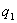and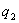be univalent in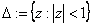with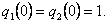We give some applications of first order differential subordination and superordination to obtain sufficient conditions for normalized analytic function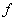with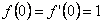to satisfy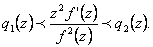26: Paper Source PDF document

Paper's Title:

A Differential Sandwich Theorem for Analytic Functions Defined by the Generalized Sălăgean Operator

Author(s):

D. Răducanu and V. O. Nechita

Faculty of Mathematics and Computer Science,
"Transilvania" University Braşov
Str. Iuliu Maniu 50, 500091 Braşov,
Romania

Faculty of Mathematics and Computer Science,
"Babeş-Bolyai" University Cluj-Napoca,
Str. M. Kogalniceanu 1, 400084 Cluj-Napoca,
Romania
URL: http://math.ubbcluj.ro/~vnechita/
vnechita@math.ubbcluj.ro

Abstract:

We obtain some subordination and superordination results involving the generalized Sălăgean differential operator for certain normalized analytic functions in the open unit disk. Our results extend corresponding previously known results.

23: Paper Source PDF document

Paper's Title:

On Stan Ulam and his Mathematics

Author(s):

Krzysztof Ciesielski and Themistocles M. Rassias

Mathematics Institute, Jagiellonian University,
Ł
jasiewicza 6, 30-348 Kraków,
Poland
Department of Mathematics. National Technical University of Athens,
Zografou Campus, 15780 Athens,
Greece

Krzysztof.Ciesielski@im.uj.edu.pl
trassias@math.ntua.gr

Abstract:

In this note we give a glimpse of the curriculum vitae of Stan Ulam, his personality and some of the mathematics he was involved in.

17: Paper Source PDF document

Paper's Title:

Inclusion and Neighborhood Properties for Certain Subclasses of Analytic Functions Associated with Convolution Structure

Author(s):

M. K. Aouf

Mathematics Department,
Faculty of Science,
Mansoura University 35516,
Egypt.
mkaouf127@yahoo.com

Abstract:

In this paper we introduce and investigate two new subclasses of multivalently analytic functions of complex order defined by using the familiar convolution structure of analytic functions. In this paper we obtain the coefficient estimates and the consequent inclusion relationships involving the neighborhoods of the p-valently analytic functions.

16: Paper Source PDF document

Paper's Title:

Action of Differential Operators On Chirpsconstruct On L

Author(s):

Taoufik El Bouayachi and Naji Yebari

Laboratoire de Mathematiques et applications,
Faculty of sciences and techniques, Tangier,
Morocco.
E-mail: figo407@gmail.com, yebarinaji@gmail.com

Abstract:

We will study in this work the action of differential operators on L chirps and we will give a new definition of logarithmic chirp. Finally we will study the action of singular integral operators on chirps by wavelet characterization and Kernel method.

14: Paper Source PDF document

Paper's Title:

Neighborhoods of Certain Subclasses of Analytic Functions of Complex Order with Negative Coefficients

Author(s):

Department of Applied Mathematics,
Sri Venkateswara College of Engineering,
Sriperumbudur, Chennai - 602105,
India.

Department of Mathematics,
Chennai - 600059,
India

Department of Applied Mathematics,
Sri Venkateswara College of Engineering,
Sriperumbudur, Chennai - 602105,
India.

Department of Mathematics,
Easwari Engineering College,
Ramapuram, Chennai - 600089,
India

Abstract:

The main object of this paper is to prove several inclusion relations associated with the (n, δ) neighborhoods of various subclasses of convex functions of complex order by making use of the known concept of neighborhoods of analytic functions.

12: Paper Source PDF document

Paper's Title:

On Singular Numbers of Hankel Matrices of Markov Functions

Author(s):

Vasily A. Prokhorov

Department of Mathematics and Statistics,
University of South Alabama,
Mobile, Alabama 36688-0002,
USA.
E-mail: prokhoro@southalabama.edu
URL: http://www.southalabama.edu/mathstat/people/prokhorov.shtml

Abstract:

Let E ⊂ (01,1) be a compact set and let μ be a positive Borel measure with support supp μ=E. Let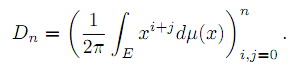In the case when E=[a,b]⊂ (-1,1) and μ satisfies the condition dμ/dx>0 a.e. on E, we investigate asymptotic behavior of singular numbers σkn,n of the Hankel matrix Dn, where kn/n→θ∈[0,1] as n→∞. Moreover, we obtain asymptotics of the Kolmogorov, Gelfand and linear k-widths, k=kn, of the unit ball An,2 of Pn∩L2(Γ) in the space L2(μ,E), where Γ={z:|z|=1} and Pn is the class of all polynomials of the degree at most n.

11: Paper Source PDF document

Paper's Title:

Some Inequalities for a Certain Class of Multivalent Functions Using Multiplier Transformation

Author(s):

Department Of Applied Mathematics
Sri Venkateswara College Of Engineering
Sriperumbudur, Chennai - 602105,
India.
suchithravenkat@yahoo.co.in

Department Of Mathematics,
Chennai - 600059,
India.

Department Of Applied Mathematics
Sri Venkateswara College Of Engineering
Sriperumbudur, Chennai - 602105,
India.
ganga@svce.ac.in

Department Of Mathematics,
Easwari Engineering College
Ramapuram, Chennai - 600089,
India.
ganga@svce.ac.in

Abstract:

The object of the present paper is to derive several inequalities associated with differential subordinations between analytic functions and a linear operator defined for a certain family of p-valent functions, which is introduced here by means of a family of extended multiplier transformations. Some special cases and consequences of the main results are also considered.

11: Paper Source PDF document

Paper's Title:

Ulam Stability of Functional Equations

Author(s):

Stefan Czerwik and Krzysztof Król

Institute of Mathematics
Silesian University of Technology
Kaszubska 23, 44-100 Gliwice,
Poland

Stefan.Czerwik@polsl.pl
Krzysztof.Krol@polsl.pl

Abstract:

In this survey paper we present some of the main results on Ulam-Hyers-Rassias stability for important functional equations.

11: Paper Source PDF document

Paper's Title:

On a Class of Meromorphic Functions of Janowski Type Related with a Convolution Operator

Author(s):

Abdul Rahman S. Juma, Husamaldin I. Dhayea

Department of Mathematics,
Iraq.
E-mail: dr_juma@hotmail.com

Department of Mathematics,
Tikrit University, Tikrit,
Iraq.

Abstract:

In this paper, we have introduced and studied new operator $Qkλ,m,γ by the Hadamard product (or convolution) of two linear operators Dkλ and Im,γ, then using this operator to study and investigate a new subclass of meromorphic functions of Janowski type, giving the coefficient bounds, a sufficient condition for a function to belong to the considered class and also a convolution property. The results presented provide generalizations of results given in earlier works. 11: Paper Source PDF document Paper's Title: Extreme Curvature of Polynomials and Level Sets Author(s): Stephanie P. Edwards, arah J. Jensen, Edward Niedermeyer, and Lindsay Willett Department of Mathematics, Hope College, Holland, MI 49423, U.S.A. E-mail: sedwards@hope.edu E-mail: tarahjaye@gmail.com E-mail: eddie.niedermeyer@gmail.com E-mail: willettlm1@gmail.com WWW: http://math.hope.edu/sedwards/ Abstract: Let f be a real polynomial of degree n. Determining the maximum number of zeros of kappa, the curvature of f, is an easy problem: since the zeros of kappa are the zeros of f'', the curvature of f is 0 at most n-2 times. A much more intriguing problem is to determine the maximum number of relative extreme values for the function kappa. Since kappa'=0 at each extreme point of kappa, we are interested in the maximum number of zeros of kappa'. In 2004, the first author and R. Gordon showed that if all the zeros of f'' are real, then f has at most n-1 points of extreme curvature. We use level curves and auxiliary functions to study the zeros of the derivatives of these functions. We provide a partial solution to this problem, showing that f has at most n-1 points of extreme curvature, given certain geometrical conditions. The conjecture that f has at most n-1 points of extreme curvature remains open. 10: Paper Source PDF document Paper's Title: A Method for Solving Systems of Nonlinear Equations Author(s): J. Shokri Department of Mathematics, Urmia University, P. O. Box 165, Urmia, Iran j.shokri@urmia.ac.ir Abstract: In this paper, we suggest and analyze a new two-step iterative method for solving nonlinear equation systems using the combination of midpoint quadrature rule and Trapezoidal quadrature rule. We prove that this method has quadratic convergence. Several examples are given to illustrate the efficiency of the proposed method. 10: Paper Source PDF document Paper's Title: Topological Aspects of Scalarization in Vector Optimization Problems. Author(s): Peter I. Kogut, Rosanna Manzo and Igor V. Nechay Department of Differential Equations, Dnipropetrovsk National University, Naukova STR., 13, 49010 Dnipropetrovsk, Ukraine p.kogut@i.ua Universitŕ di Salerno, Dipartimento di Ingegneria dell'Informazione e Matematica Applicata, Via Ponte don Melillo, 84084 Fisciano (SA), Italy manzo@diima.unisa.it Department of Technical Cybernetics, Dnipropetrovsk Technical University, Acad. Lazarjan STR., 2, 49010 Dnipropetrovsk, Ukraine i.nechay@i.ua Abstract: In this paper, we study vector optimization problems in partially ordered Banach spaces. We suppose that the objective mapping possesses a weakened property of lower semicontinuity and make no assumptions on the interior of the ordering cone. We derive sufficient conditions for existence of efficient solutions of the above problems and discuss the role of topological properties of the objective space. We discuss the scalarization of vector optimization problems when the objective functions are vector-valued mappings with a weakened property of lower semicontinuity. We also prove the existence of the so-called generalized efficient solutions via the scalarization process. All principal notions and assertions are illustrated by numerous examples. 8: Paper Source PDF document Paper's Title: Subordination Results Associated with Hadamard Product Author(s): S. Sivasubramanian, C. Ramachandran and B. A. Frasin Department of Mathematics, University College of Engineering, Anna University, Saram-604 307, India sivasaisastha@rediffmail.com Department of Mathematics, University College of Engineering, Anna University, Villupuram, India crjsp2004@yahoo.com Department of Mathematics, Al al-Bayt University, P.O. Box: 130095 Mafraq, Jordan Abstract: In the present investigation, we consider an unified class of functions of complex order using Hadamard's convolution. We obtain a necessary and sufficient condition for functions to be in these classes. 8: Paper Source PDF document Paper's Title: Certain Coefficient Estimates for Bi-univalent Sakaguchi Type Functions Author(s): B. Srutha Keerthi, S. Chinthamani Department of Applied Mathematics, Sri Venkateswara College of Engineering, Sriperumbudur, Chennai - 602105, India Abstract: Estimates on the initial coefficients are obtained for normalized analytic functions f in the open unit disk with f and its inverse g = f-1 satisfying the conditions that zf'(z) / f(z) and zg'(z) / g(z) are both subordinate to a starlike univalent function whose range is symmetric with respect to the real axis. Several related classes of functions are also considered, and connections to earlier known results are made. 8: Paper Source PDF document Paper's Title: Properties of Certain Multivalent Functions Involving Ruscheweyh Derivatives Author(s): N-Eng Xu and Ding-Gong Yang Department of Mathematics, Changshu Institute of Technology, Changshu, Jiangsu 215500, China Abstract: Let Ap(p∈ N) be the class of functions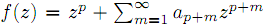which are analytic in the unit disk. By virtue of the Ruscheweyh derivatives we introduce the new subclasses Cp(n,α,β,λ,μ) of Ap. Subordination relations, inclusion relations, convolution properties and a sharp coefficient estimate are obtained. We also give a sufficient condition for a function to be in Cp(n,α,β,λ,μ) 8: Paper Source PDF document Paper's Title: A Subordination Theorem for Analytic Functions Author(s): Marjono Department of Mathematics, Faculty of Mathematics and Natural Sciences, University of Brawijaya, Jl. Veteran Malang 65145, INDONESIA. E-mail: marjono@ub.ac.id URL: http://matematika.ub.ac.id Abstract: It is shown that if f is analytic in D={z:|z|<1}, with f(0)=f'(0)-1=0, then for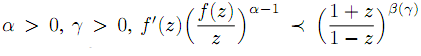implies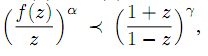where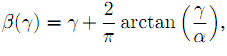and that β(γ) is the largest number such that this implication holds. 8: Paper Source PDF document Paper's Title: Countable Ordinal Spaces and Compact Countable Subsets of a Metric Space Author(s): B. Alvarez-Samaniego, A. Merino Nucleo de Investigadores Cientificos Facultad de Ciencias, Universidad Central del Ecuador (UCE) Quito, Ecuador. E-mail: borys_yamil@yahoo.com, balvarez@uce.edu.ec Escuela de Ciencias Fisicas y Matematica Facultad de Ciencias Exactas y Naturales Pontificia Universidad Catolica del Ecuador Apartado: 17-01-2184, Quito, Ecuador. E-mail: aemerinot@puce.edu.ec Abstract: We show in detail that every compact countable subset of a metric space is homeomorphic to a countable ordinal number, which extends a result given by Mazurkiewicz and Sierpinski for finite-dimensional Euclidean spaces. In order to achieve this goal, we use Transfinite Induction to construct a specific homeomorphism. In addition, we prove that for all metric space, the cardinality of the set of all the equivalence classes, up to homeomorphisms, of compact countable subsets of this metric space is less than or equal to aleph-one. We also show that for all cardinal number smaller than or equal to aleph-one, there exists a metric space with cardinality equals the aforementioned cardinal number. 8: Paper Source PDF document Paper's Title: Coefficient Estimates Of Sakaguchi Kind Functions Using Lucas Polynomials Author(s): H. Priya and B. Srutha Keerthi Department of Mathematics, School of Advanced Sciences, VIT Chennai Campus, Chennai - 600 048, India. E-mail: priyaharikrishnan18@gmail.com Department of Mathematics, School of Advanced Sciences, VIT Chennai Campus, Chennai - 600 048, India. E-mail: i sruthilaya06@yahoo.co.in Abstract: By means of (p,q) Lucas polynomials, we estimate coefficient bounds and Fekete-Szego inequalities for functions belonging to this class. Several corollaries and consequences of the main results are also obtained. 7: Paper Source PDF document Paper's Title: On a Criteria for Strong Starlikeness Author(s): V. Ravichandran, M. Darus, and N. Seenivasagan School Of Mathematical Sciences, Universiti Sains Malaysia, 11800 Usm Penang, Malaysia vravi@cs.usm.my URL: http://cs.usm.my/~vravi School of Mathematical Sciences, Faculty of Sciences and Technology, Ukm, Bangi 43600, Malaysia maslina@pkrisc.cc.ukm.my Sindhi College, 123, P. H. Road, Numbal, Chennai 600 077 India vasagan2000@yahoo.co.in Abstract: In this paper, we are concerned with finding sufficient condition for certain normalized analytic function f(z) defined on the open unit disk in the complex plane to be strongly starlike of order α. Also we have obtained similar results for certain functions defined by Ruscheweyh derivatives and Sălăgean derivatives. Further extension of these results are given for certain p-valent analytic functions defined through a linear operator. 7: Paper Source PDF document Paper's Title: Komatu Integral Transforms of Analytic Functions Subordinate to Convex Functions Author(s): T. N. Shanmugam and C. Ramachandran Department of Mathematics, College of Engineering, Anna University, Chennai-600 025, Tamilnadu, India shan@annauniv.edu Department of Mathematics, College of Engineering, Anna University, Chennai-600 025, Tamilnadu, India crjsp2004@yahoo.com Abstract: In this paper, we consider the class A of the functions f(z) of the form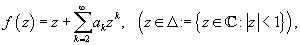which are analytic in an open disk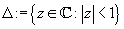and study certain subclass of the class A, for which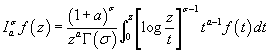has some property. Certain inclusion and the closure properties like convolution with convex univalent function etc. are studied. 7: Paper Source PDF document Paper's Title: Hyperbolic Barycentric Coordinates Author(s): Abraham A. Ungar Department of Mathematics, North Dakota State University, Fargo, ND 58105, USA Abraham.Ungar@ndsu.edu URL : http://math.ndsu.nodak.edu/faculty/ungar/ Abstract: A powerful and novel way to study Einstein's special theory of relativity and its underlying geometry, the hyperbolic geometry of Bolyai and Lobachevsky, by analogies with classical mechanics and its underlying Euclidean geometry is demonstrated. The demonstration sets the stage for the extension of the notion of barycentric coordinates in Euclidean geometry, first conceived by Möbius in 1827, into hyperbolic geometry. As an example for the application of hyperbolic barycentric coordinates, the hyperbolic midpoint of any hyperbolic segment, and the centroid and orthocenter of any hyperbolic triangle are determined. 6: Paper Source PDF document Paper's Title: An Application of Quasi Power Increasing Sequences Author(s): Hüseyín Bor Department of Mathematics, Erciyes University, 38039 Kayseri, Turkey rl: Abstract: In this paper a result of Bor  has been proved under weaker conditions by using a -quasi power increasing sequence instead of an almost increasing sequence. 6: Paper Source PDF document Paper's Title: Construction of a Frame Multiresolution Analysis on Locally Compact Abelian Groups Author(s): R. Kumar and Satyapriya Department of Mathematics, Kirori Mal College, University of Delhi, Delhi, India. E-mail: rajkmc@gmail.com Department of Mathematics, University of Delhi, Delhi, India. E-mail: kmc.satyapriya@gmail.com Abstract: The frame multiresolution analysis (FMRA) on locally compact Abelian groups has been studied and the results concerning classical MRA have been worked upon to obtain new results. All the necessary conditions, which need to be imposed on the scaling function φ to construct a wavelet frame via FMRA, have been summed up. This process of construction of FMRA has aptly been illustrated by sufficient examples. 5: Paper Source PDF document Paper's Title: An Algorithm to Compute Gaussian-Type Quadrature Formulae that Integrate Polynomials and Some Spline Functions Exactly Author(s): Allal Guessab Laboratoire de Mathématiques Appliquées, Université de Pau, 64000, Pau, France. allal.guessab@univ-pau.fr URL: http://www.univ-pau.fr/~aguessab/ Abstract: It is well-known that for sufficiently smooth integrands on an interval, numerical integration can be performed stably and efficiently via the classical (polynomial) Gauss quadrature formulae. However, for many other sets of integrands these quadrature formulae do not perform well. A very natural way of avoiding this problem is to include a wide class among arbitrary functions (not necessary polynomials) to be integrated exactly. The spline functions are natural candidates for such problems. In this paper, after studying Gaussian type quadrature formulae which are exact for spline functions and which contain boundary terms involving derivatives at both end points, we present a fast algorithm for computing their nodes and weights. It is also shown, taking advantage of the close connection with ordinary Gauss quadrature formula, that the latter are computed, via eigenvalues and eigenvectors of real symmetric tridiagonal matrices. Hence a new class of quadrature formulae can then be computed directly by standard software for ordinary Gauss quadrature formula. Comparative results with classical Gauss quadrature formulae are given to illustrate the numerical performance of the approach. 5: Paper Source PDF document Paper's Title: A general theory of decision making Author(s): Frank Hansen Department of Economics, University of Copenhagen, Studiestraede 6, DK-1455 Copenhagen K Denmark Frank.Hansen@econ.ku.dk URL: http://www.econ.ku.dk/okofh Abstract: We formulate a general theory of decision making based on a lattice of observable events, and we exhibit a large class of representations called the general model. Some of the representations are equivalent to the so called standard model in which observable events are modelled by an algebra of measurable subsets of a state space, while others are not compatible with such a description. We show that the general model collapses to the standard model, if and only if an additional axiom is satisfied. We argue that this axiom is not very natural and thus assert that the standard model may not be general enough to model all relevant phenomena in economics. Using the general model we are (as opposed to Schmeidler ) able to rationalize Ellsberg's paradox without the introduction of non-additive measures. 5: Paper Source PDF document Paper's Title: Reconstruction of Discontinuities of Functions Given Noisy Data Author(s): Eric D. Mbakop 67A Beaver Park Rd, Framingham, MA, 01702, U. S. A. ericsteve86@yahoo.fr Abstract: Suppose one is given noisy data of a discontinuous piecewise-smooth function along with a bound on its second derivative. The locations of the points of discontinuity of f and their jump sizes are not assumed known, but are instead retrieved stably from the noisy data. The novelty of this paper is a numerical method that allows one to locate some of these points of discontinuity with an accuracy that can be made arbitrarily small. 5: Paper Source PDF document Paper's Title: Fekete-Szegö Problem for Univalent Functions with Respect to k-Symmetric Points Author(s): K. Al-Shaqsi and M. Darus School of Mathematical Sciences, Faculty of Science and Technology, University Kebangsaan Malaysia, Bangi 43600 Selangor D. Ehsan, Malaysia ommath@hotmail.com maslina@ukm.my Abstract: In the present investigation, sharp upper bounds of |a3- μa22| for functions f(z) = z + a2z2 + a2z3 + ... belonging to certain subclasses of starlike and convex functions with respect to k-symmetric points are obtained. Also certain applications of the main results for subclasses of functions defined by convolution with a normalized analytic function are given. In particular, Fekete- Szegö inequalities for certain classes of functions defined through fractional derivatives are obtained. 5: Paper Source PDF document Paper's Title: A Subclass of Meromorphically Multivalent Functions with Applications to Generalized Hypergeometric Functions Author(s): M. K. Aouf Mathematics Department, Faculty of Science, Mansoura University 35516, Egypt mkaouf127@yahoo.com Abstract: In this paper a new subclass of meromorphically multivalent functions, which is defined by means of a Hadamard product (or convolution) involving some suitably normalized meromorphically p-valent functions. The main object of the present paper is to investigate the various important properties and characteristics of this subclass of meromorphically multivalent functions. We also derive many interesting results for the Hadamard products of functions belonging to this subclass. Also we consider several applications of our main results to generalized hypergeomtric functions. 5: Paper Source PDF document Paper's Title: On the Convergence in Law of Iterates of Random-Valued Functions Author(s): Karol Baron Uniwersytet Śląski, Instytut Matematyki Bankowa 14, PL--40--007 Katowice, Poland baron@us.edu.pl Abstract: Given a probability space (Ω, A, P) a separable and complete metric space X with the σ-algebra B of all its Borel subsets and a B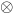A -measurable f : X * Ω → X we consider its iterates fn, n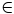N, defined on X * ΩN by f1(x,ω) = f(x,ω1) and fn+1(x,ω)=f(fn(x,ω),ωn+1), provide a simple criterion for the convergence in law of fn(x,·)) nN, to a random variable independent of xX , and apply this criterion to linear functional equations in a single variable. 5: Paper Source PDF document Paper's Title: Shape Diagrams for 2D Compact Sets - Part I: Analytic Convex Sets. Author(s): S. Rivollier, J. Debayle and J.-C. Pinoli Ecole Nationale Supérieure des Mines de Saint-Etienne, CIS - LPMG, UMR CNRS 5148, 158 cours Fauriel, 42023 Saint-Etienne Cedex 2, France. Abstract: Shape diagrams are representations in the Euclidean plane introduced to study 3-dimensional and 2-dimensional compact convex sets. Such a set is represented by a point within a shape diagram whose coordinates are morphometrical functionals defined as normalized ratios of geometrical functionals. Classically, the geometrical functionals are the area, the perimeter, the radii of the inscribed and circumscribed circles, and the minimum and maximum Feret diameters. They allow thirty-one shape diagrams to be built. Most of these shape diagrams can also been applied to more general compact sets than compact convex sets. Starting from these six classical geometrical functionals, a detailed comparative study has been performed in order to analyze the representation relevance and discrimination power of these thirty-one shape diagrams. The purpose of this paper is to present the first part of this study, by focusing on analytic compact convex sets. A set will be called analytic if its boundary is piecewise defined by explicit functions in such a way that the six geometrical functionals can be straightforwardly calculated. The second and third part of the comparative study are published in two following papers [19.20]. They are focused on analytic simply connected sets and convexity discrimination for analytic and discretized simply connected sets, respectively. 5: Paper Source PDF document Paper's Title: Inclusion Properties of a Certain Subclass of Strongly Close-To-Convex Functions Author(s): S. M. Khairnar and M. More Department of Mathematics, Maharashtra Academy of Engineerring, Alandi -412 105, Pune, Maharashtra, INDIA. smkhairnar2007@gmail.com, meenamores@gmail.com. Abstract: The purpose of this paper is to derive some inclusion and argument properties of a new subclass of strongly close-to-convex functions in the open unit disc. We have considered an integral operator defined by convolution involving hypergeometric function in the subclass definition. The subclass also extends to the class of α-spirallike functions of complex order. 5: Paper Source PDF document Paper's Title: Sharp Lp Improving Results for Singular Measures on Cn+1 Author(s): E. Ferreyra, M. Urciuolo FaMAF-CIEM, Universidad Nacional de Córdoba-Conicet, Ciudad Universitaria, 5000 Córdoba, Argentina Abstract: For j=1,...,n, let Ωj be open sets of the complex plane and let φj be holomorphic functions on Ωj such that φj'' does not vanish identically on Ωj. We consider φ(z1,...,zn) =φ1(z1) +...+φn(zn). We characterize the pairs (p,q) such that the convolution operator with the surface measure supported on a compact subset of the graph of φ is p-q bounded. 5: Paper Source PDF document Paper's Title: Fractional class of analytic functions Defined Using q-Differential Operator Author(s): K . R. Karthikeyan, Musthafa Ibrahim and S. Srinivasan Department of Mathematics and Statistics, Caledonian College of Engineering, Muscat, Sultanate of Oman. E-mail: kr_karthikeyan1979@yahoo.com College of Engineering, University of Buraimi, Al Buraimi, Sultanate of Oman. E-mail: musthafa.ibrahim@gmail.com Department of Mathematics, Presidency College (Autonomous), Chennai-600005, Tamilnadu, India. Abstract: We define a q-differential fractional operator, which generalizes Salagean and Ruscheweyh differential operators. We introduce and study a new class of analytic functions involving q-differential fractional operator. We also determine the necessary and sufficient conditions for functions to be in the class. Further, we obtain the coefficient estimates, extreme points, growth and distortion bounds. 4: Paper Source PDF document Paper's Title: Generalizations of two theorems on absolute summability methods Author(s): H.S. Özarslan and H.N. Öğdük Department of Mathematics, Erciyes University, 38039 Kayseri, Turkey seyhan@erciyes.edu.tr nogduk@erciyes.edu.tr URL: http://fef.erciyes.edu.tr/math/hikmet.htm Abstract: In this paper two theorems on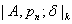summability methods, which generalize two theorems of Bor on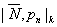summability methods, have been proved. 4: Paper Source PDF document Paper's Title: Distortion Theorems for Certain Analytic Functions Involving the Coefficient Inequalities Author(s): Shigeyoshi Owa and Junichi Nishiwaki Department of Mathematics, Kinki University, Higashi-Osaka, Osaka 577-8502, Japan Abstract: By virtue of the coefficient inequalities for certain analytic functions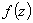in the open unit disk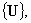two subclasses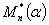and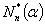are introduced. The object of the present paper is to discuss the distortion thorems of functionsbelonging to the classesinvolving the coefficient inequalities. 4: Paper Source PDF document Paper's Title: On Some Ramanujan's Schläfli Type Modular Equations Author(s): K. R. Vasuki Department of Mathematics, Acharya Institute of Technology, Soldevanahalli, Chikkabanavara (Post), Hesaragatta Main Road, Bangalore-560 090, INDIA. vasuki_kr@hotmail.com Abstract: In this paper, we give new proof of certain Ramanujan-Schläfli modular equations. We also obtain a new modular equation of degree 23. 4: Paper Source PDF document Paper's Title: On a Method of Proving the Hyers-Ulam Stability of Functional Equations on Restricted Domains Author(s): Janusz Brzdęk Department of Mathematics Pedagogical University Podchor ąźych 2, 30-084 Krak ów, Poland jbrzdek@ap.krakow.pl Abstract: We show that generalizations of some (classical) results on the Hyers-Ulam stability of functional equations, in several variables, can be very easily derived from a simple result on stability of a functional equation in single variable 4: Paper Source PDF document Paper's Title: On the product of M-measures in l-groups Author(s): A. Boccuto, B. Riěcan, and A. R. Sambucini Dipartimento di Matematica e Informatica, via Vanvitelli, 1 I-06123 Perugia, Italy. boccuto@dipmat.unipg.it URL: http://www.dipmat.unipg.it/~boccuto Katedra Matematiky, Fakulta Prírodných Vied, Univerzita Mateja Bela, Tajovského, 40, Sk-97401 Banská Bystrica, Slovakia. riecan@fpv.umb.sk Dipartimento di Matematica e Informatica, via Vanvitelli, 1 I-06123 Perugia, Italy. matears1@unipg.it URL: http://www.unipg.it/~matears1 Abstract: Some extension-type theorems and compactness properties for the product of l-group-valued M-measures are proved. 4: Paper Source PDF document Paper's Title: Generalized Efficient Solutions to One Class of Vector Optimization Problems in Banach Space Author(s): Peter I. Kogut, Rosanna Manzo, and Igor V. Nechay Department of Differential Equations, Dnipropetrovsk National University, Naukova str., 13, 49050 Dnipropetrovsk, Ukraine p.kogut@i.ua Dipartimento di Ingegneria Dell’informazione e Matematica Applicata, Universitŕ di Salerno, Via Ponte Don Melillo, 84084 Fisciano (Sa), Italy manzo@diima.unisa.it Department of Technical Cybernetics, Dnipropetrovsk Technical University, Acad. Lazarjan str., 2, 49010 Dnipro petrovsk, Ukraine i.nechay@i.ua Abstract: In this paper, we study vector optimization problems in Banach spaces for essentially nonlinear operator equations with additional control and state constraints. We assume that an objective mapping possesses a weakened property of lower semicontinuity and make no assumptions on the interior of the ordering cone. Using the penalization approach we derive both sufficient and necessary conditions for the existence of efficient solutions of the above problems. We also prove the existence of the so-called generalized efficient solutions via the scalarization of some penalized vector optimization problem. 4: Paper Source PDF document Paper's Title: Shape Diagrams for 2D Compact Sets - Part II: Analytic Simply Connected Sets. Author(s): S. Rivollier, J. Debayle and J.-C. Pinoli Ecole Nationale Supérieure des Mines de Saint-Etienne, CIS - LPMG, UMR CNRS 5148, 158 cours Fauriel, 42023 Saint-Etienne Cedex 2, France. Abstract: Shape diagrams are representations in the Euclidean plane introduced to study 3-dimensional and 2-dimensional compact convex sets. However, they can also been applied to more general compact sets than compact convex sets. A compact set is represented by a point within a shape diagram whose coordinates are morphometrical functionals defined as normalized ratios of geometrical functionals. Classically, the geometrical functionals are the area, the perimeter, the radii of the inscribed and circumscribed circles, and the minimum and maximum Feret diameters. They allow twenty-two shape diagrams to be built. Starting from these six classical geometrical functionals, a detailed comparative study has been performed in order to analyze the representation relevance and discrimination power of these twenty-two shape diagrams. The first part of this study is published in a previous paper 16. It focused on analytic compact convex sets. A set will be called analytic if its boundary is piecewise defined by explicit functions in such a way that the six geometrical functionals can be straightforwardly calculated. The purpose of this paper is to present the second part, by focusing on analytic simply connected compact sets. The third part of the comparative study is published in a following paper 17. It is focused on convexity discrimination for analytic and discretized simply connected compact sets. 4: Paper Source PDF document Paper's Title: On Some Constructive Method of Rational Approximation Author(s): Vasiliy A. Prokhorov Department of Mathematics and Statistics University of South Alabama Mobile, Alabama 36688-0002, USA. Abstract: We study a constructive method of rational approximation of analytic functions based on ideas of the theory of Hankel operators. Some properties of the corresponding Hankel operator are investigated. We also consider questions related to the convergence of rational approximants. Analogues of Montessus de Ballore's and Gonchars's theorems on the convergence of rows of Padé approximants are proved. 4: Paper Source PDF document Paper's Title: A Note on Divergent Fourier Series and λ-Permutations Author(s): A. Castillo, J. Chavez and H. Kim Tufts University, Department of Mathematics, Medford, MA 02155, USA E-mail: angel.castillo@tufts.edu Texas Tech University, Department of Mathematics and Statistics, Lubbock, TX 79409, USA E-mail: josechavez5@my.unt.edu University of Michigan-Dearborn, Department of Mathematics and Statistics, Dearborn, MI 48128, USA. E-mail: khyejin@umich.edu Abstract: We present a continuous function on [-π,π] whose Fourier series diverges and it cannot be rearranged to converge by a λ-permutation. 3: Paper Source PDF document Paper's Title: On the Ulam Stability for Euler-Lagrange Type Quadratic Functional Equations Author(s): Matina John Rassias and John Michael Rassias Statistics and Modelling Science, University of Strathclyde, Livingstone Tower, 26 Richmond Str, Glasgow, Uk, G1 1xh Pedagogical Department, E. E., National and Capodistrian University of Athens, Section of Mathematics and Informatics, 4, Agamemnonos Str, Aghia Paraskevi, Athens 15342, Greece Abstract: In 1940 (and 1968) S. M. Ulam proposed the well-known Ulam stability problem. In 1941 D.H. Hyers solved the Hyers-Ulam problem for linear mappings. In 1951 D. G. Bourgin has been the second author treating the Ulam problem for additive mappings. In 1978 according to P.M. Gruber this kind of stability problems is of particular interest in probability theory and in the case of functional equations of different types. In 1982-2004 we established the Hyers-Ulam stability for the Ulam problem for different mappings. In 1992-2000 J.M. Rassias investigated the Ulam stability for Euler-Lagrange mappings. In this article we solve the Ulam problem for Euler-Lagrange type quadratic functional equations. These stability results can be applied in mathematical statistics, stochastic analysis, algebra, geometry, as well as in psychology and sociology. 3: Paper Source PDF document Paper's Title: Solution of the Hyers-Ulam Stability Problem for Quadratic Type Functional Equations in Several Variables Author(s): John Michael Rassias Pedagogical Department, E.E., National and Capodistrian University of Athens, Section of Mathematics and Informatics, 4, Agamemnonos Str., Aghia Paraskevi, Athens 15342, Greece jrassias@primedu.uoa.gr URL: http://www.primedu.uoa.gr/~jrassias/ Abstract: In 1940 (and 1968) S. M. Ulam proposed the well-known Ulam stability problem. In 1941 D. H. Hyers solved the Hyers-Ulam problem for linear mappings. In 1951 D. G. Bourgin has been the second author treating the Ulam problem for additive mappings. In 1978 according to P. M. Gruber this kind of stability problems is of particular interest in probability theory and in the case of functional equations of different types. In 1982-2004 we established the Hyers-Ulam stability for the Ulam problem for different mappings. In this article we solve the Hyers-Ulam problem for quadratic type functional equations in several variables. These stability results can be applied in stochastic analysis, financial and actuarial mathematics, as well as in psychology and sociology. 3: Paper Source PDF document Paper's Title: On the Generalized Stability and Asymptotic Behavior of Quadratic Mappings Author(s): Hark-Mahn Kim, Sang-Baek Lee and Eunyoung Son Department of Mathematics Chungnam National University Daejeon, 305-764, Republic of Korea hmkim@cnu.ac.kr Abstract: We extend the stability of quadratic mappings to the stability of general quadratic mappings with several variables, and then obtain an improved asymptotic property of quadratic mappings on restricted domains. 3: Paper Source PDF document Paper's Title: Purely Unrectifiable Sets with Large Projections Author(s): Harold R. Parks Department of Mathematics, Oregon State University, Corvallis, Oregon 97331--4605, USA parks@math.oregonstate.edu URL : http://www.math.oregonstate.edu/people/view/parks/ Abstract: For n2, we give a construction of a compact subset of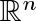that is dispersed enough that it is purely unrectifiable, but that nonetheless has an orthogonal projection that hits every point of an (n-1)-dimensional unit cube. Moreover, this subset has the additional surprising property that the orthogonal projection onto any straight line inis a set of positive 1-dimensional Hausdorff measure. 3: Paper Source PDF document Paper's Title: Hyers-Ulam-Rassias Stability of a Generalized Jensen Functional Equation Author(s): A. Charifi, B. Bouikhalene, E. Elqorachi and A. Redouani Department of Mathematics, Faculty of Sciences, Ibn Tofail University, Kenitra, Morocco charifi2000@yahoo.fr bbouikhalene@yahoo.fr Department of Mathematics, Faculty of Sciences, Ibn Zohr University, Agadir, Morocco elqorachi@hotmail.com Redouani-ahmed@yahoo.fr Abstract: In this paper we obtain the Hyers-Ulam-Rassias stability for the generalized Jensen's functional equation in abelian group (G,+). Furthermore we discuss the case where G is amenable and we give a note on the Hyers-Ulam-stability of the K-spherical (n × n)-matrix functional equation. 3: Paper Source PDF document Paper's Title: A New Method for Comparing Closed Intervals Author(s): Ibraheem Alolyan Department of Mathematics, College of Sciences, King Saud University, P. O. Box 2455, Riyadh 11451, Saudi Arabia ialolyan@ksu.edu.sa URL:http://faculty.ksu.edu.sa/ALolyan Abstract: The usual ordering ≤" on R is a total ordering, that is, for any two real numbers in R, we can determine their order without difficulty. However, for any two closed intervals in R, there is not a natural ordering among the set of all closed intervals in R. Several methods have been developed to compare two intervals. In this paper, we define the μ-ordering which is a new method for ordering closed intervals. 3: Paper Source PDF document Paper's Title: Bartle Integration in Lie Algebras Author(s): Andreas Boukas and Philip Feinsilver Centro Vito Volterra, Universita di Roma Tor Vergata, via Columbia 2, 00133 Roma, Italy. Department of Mathematics, Southern Illinois University, Carbondale, Illinois 62901, USA. E-mail: andreasboukas@yahoo.com E-mail: pfeinsil@math.siu.edu Abstract: Using Bartle's bilinear vector integral we define stochastic integrals of bounded operator valued functions with respect to Stieltjes measures associated with the generators of the Heisenberg and Finite Difference Lie algebras. Our definition also covers the Square of White Noise and sl/2 Lie algebras. 3: Paper Source PDF document Paper's Title: Stability of the D-Bar Reconstruction Method for Complex Conductivities Author(s): 1S. El Kontar, 1T. El Arwadi, 1H. Chrayteh, 2J.-M. Sac-Épée 1Department of Mathematics and Computer Science, Faculty of Science, Beirut Arab University, P.O. Box: 11-5020, Beirut, Lebanon. E-mail: srs915@student.bau.edu.lb 2Institut Élie Cartan de Lorraine, Université de Lorraine - Metz, France. Abstract: In 2000, Francini solved the inverse conductivity problem for twice-differentiable conductivities and permittivities. This solution was considered to be the first approach using D-bar methods with complex conductivities. In 2012, based on Francini's work, Hamilton introduced a reconstruction method of the conductivity distribution with complex values. The method consists of six steps. A voltage potential is applied on the boundary. Solving a D-Bar equation gives the complex conductivity. In this paper, the stability of the D-Bar equation is studied via two approximations, texp and tB, for the scattering transform. The study is based on rewriting the reconstruction method in terms of continuous operators. The conductivity is considered to be non smooth. 3: Paper Source PDF document Paper's Title: The Dynamics of an Ebola Epidemic Model with Quarantine of Infectives Author(s): Eliab Horub Kweyunga Department of Mathematics, Kabale University, P.O.Box 317, Kabale, Uganda. E-mail: hkweyunga@kab.ac.ug Abstract: The recurrent outbreaks of ebola in Africa present global health challenges. Ebola is a severe, very fatal disease with case fatality rates of up to 90%. In this paper, a theoretical deterministic model for ebola epidemic with quarantine of infectives is proposed and analyzed. The model exhibits two equilibria; the disease free and endemic equilibrium points. The basic reproduction number, R0, which is the main threshold, is obtained and the stability of the equilibrium points established. Using parameter values drawn from the 2014 West Africa ebola outbreak, a numerical simulation of the model is carried out. It is found that the dynamics of the model are completely determined by R0 and that a quarantine success rate of at least 70% is sufficient to contain the disease outbreak. 3: Paper Source PDF document Paper's Title: Euler-Maclaurin Formulas for Functions of Bounded Variation Author(s): G. De Marco, M. De Zotti, C. Mariconda Dipartimento di Matematica Tullio Levi-Civita, Universita degli Studi di Padova Via Trieste 63, Padova 35121, Italy. E-mail: carlo.mariconda@unipd.it URL: http://www.math.unipd.it Abstract: The first-order Euler-Maclaurin formula relates the sum of the values of a smooth function on an interval of integers with its integral on the same interval on R. We formulate here the analogue for functions that are just of bounded variation. 3: Paper Source PDF document Paper's Title: On a subset of Bazilevic functions Author(s): Marjono and D. K. Thomas Department of Mathematics, Faculty of Mathematics and Natural Sciences, Brawajaya University, Malang, Jawa Timur 65145, Indonesia. E-mail: marjono@ub.ac.id Department of Mathematics, Swansea University, Singleton Park, Swansea, SA2 8PP, United Kingdom. E-mail: d.k.thomas@swansea.ac.uk Abstract: Let S denote the class of analytic and univalent functions in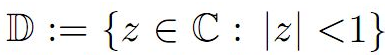of the form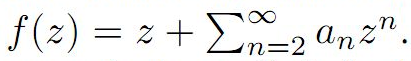For α≥0, the subclass B1α of S of Bazilevic functions has been extensively studied. In this paper we determine various properties of a subclass of B1α, for α≥0 which extends early results of a class of starlike functions studied by Ram Singh. 3: Paper Source PDF document Paper's Title: Existence of Solution of Differential and Riemann-Liouville Equation Via Fixed Point Approach in Complex Valued b-Metric Spaces Author(s): K. Afassinou, A. A. Mebawondu, H. A. Abass and O. K. Narain Department of Science Access, University of Zululand, KwaDlangezwa, South Africa. E-mail: komia@aims.ac.za DST-NRF Centre of Excellence in Mathematical and Statistical Sciences (CoE-MaSS), Johannesburg, South Africa. E-mail: dele@aims.ac.za DST-NRF Centre of Excellence in Mathematical and Statistical Sciences (CoE-MaSS), Johannesburg, South Africa. E-mail: hammedabass548@gmail.com School of Mathematics, Statistics and Computer Science, University of KwaZulu-Natal, Durban, South Africa. E-mail: naraino@ukzn.ac.za Abstract: In this paper, we establish some fixed point and common fixed point results for a new type of generalized contractive mapping using the notion of C-class function in the framework of complex valued b-metric spaces. As an application, we establish the existence and uniqueness of a solution for Riemann-Liouville integral and ordinary differential equation in the framework of a complete complex valued b-metric spaces. The obtained results generalize and improve some fixed point results in the literature. 2: Paper Source PDF document Paper's Title: Asymptotic Behavior of Mixed Type Functional Equations Author(s): J. M. Rassias Pedagogical Department, E.E., National and Capodistrian University of Athens, Section of Mathematics And Informatics, 4, Agamemnonos Str., Aghia Paraskevi, Athens 15342,Greece jrassias@primedu.uoa.gr URL: http://www.primedu.uoa.gr/~jrassias/ Abstract: In 1983 Skof  was the first author to solve the Ulam problem for additive mappings on a restricted domain. In 1998 Jung  investigated the Hyers-Ulam stability of additive and quadratic mappings on restricted domains. In this paper we improve the bounds and thus the results obtained by Jung , in 1998 and by the author , in 2002. Besides we establish new theorems about the Ulam stability of mixed type functional equations on restricted domains. Finally, we apply our recent results to the asymptotic behavior of functional equations of different types. 2: Paper Source PDF document Paper's Title: Essential Random Fixed Point Set of Random Operators Author(s): Ismat Beg Centre for Advanced Studies in Mathematics, Lahore University of Management Sciences (LUMS), 54792-Lahore, PAKISTAN. ibeg@lums.edu.pk URL: http://web.lums.edu.pk/~ibeg Abstract: We obtain necessary and sufficient conditions for the existence of essential random fixed point of a random operator defined on a compact metric space. The structure of the set of essential random fixed points is also studied. 2: Paper Source PDF document Paper's Title: A relation between nuclear cones and full nuclear cones Author(s): G. Isac and A. B. Nemeth Department of Mathematics, Royal Military College of Canada, P. O. Box 17000 STN Forces Kingston, Ontario, Canada K7K 7B4. isac-g@rmc.ca Faculty of Mathematics and Computer Science, Babes-Bolyai University, 3400 Cluj-Napoca, Romania. nemab@math.ubbcluj.ro Abstract: The notion of nuclear cone in locally convex spaces corresponds to the notion of well based cone in normed spaces. Using the bipolar theorem from locally convex spaces it is proved that every closed nuclear cone is a full nuclear cone. Thus every closed nuclear cone can be associated to a mapping from a family of continuous seminorms in the space to the topological dual of the space. The relation with Pareto efficiency is discussed. 2: Paper Source PDF document Paper's Title: Analysis of the Flow Field in Stenosed Bifurcated Arteries Through a Mathematical Model Author(s): S. Chakravarty and S. Sen Department of Mathematics, Visva-Bharati University, Santiniketan 731235, India santabrata2004@yahoo.co.in Abstract: The present study is dealt with an appropriate mathematical model of the arotic bifurcation in the presence of constrictions using which the physiological flow field is analized. The geometry of the bifurcated arterial segment having constrictions in both the parent and its daughter arterial lumen frequently occurring in the diseased arteries causing malfunction of the cardiovascular system , is formed mathematically with the introduction of appropriate curvatures at the lateral junctions and the flow divider. The flowing blood contained in the stenosed bifurcated artery is treated to be Newtonian and the flow is considered to be two dimensional. The motion of the arterial wall and its effect on local fluid mechanics is not ruled out from the present pursuit. The flow analysis applies the time-dependent, two-dimensional incompressible nonlinear Navier-Stokes equations for Newtonian fluid. The flow field can be obtained primarily following the radial coordinate transformation and using the appropriate boundary conditions and finally adopting a suitable finite difference scheme numerically. The influences of the arterial wall distensibility and the presence of stenosis on the flow field, the flow rate and the wall shear stresses are quantified in order to indicate the susceptibility to atherosclerotic lesions and thereby to validate the applicability of the present theoretical model. 2: Paper Source PDF document Paper's Title: On the Fekete-Szegő Inequality for Some Subclasses of Analytic Functions Author(s): T.N. Shanmugam and A. Singaravelu Department of Mathematics, College of Engineering, Anna University, Chennai-600 025, Tamilnadu, India shan@annauniv.edu Department of Mathematics, Valliammai Engineering College, Chennai-603 203, Tamilnadu, India sivasaisastha@rediffmail.com Abstract: In this present investigation, the authors obtainFekete-Szegő's inequality for certain normalized analytic functionsdefined on the open unit disk for which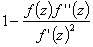lie in a region starlike with respect to 1 and symmetric with respect to the real axis. Also certain applications of the main result for a class of functions defined by convolution are given. As a special case of this result, Fekete-Szegő's inequality for a class of functions defined through fractional derivatives is also obtained. 2: Paper Source PDF document Paper's Title: 1-type Pseudo-Chebyshev Subspaces in Generalized 2-normed Spaces Author(s): Sh. Rezapour Department of Mathematics, Azarbaijan University of Tarbiat Moallem, Azarshahr, Tabriz, Iran sh.rezapour@azaruniv.edu Abstract: We construct a generalized 2-normed space from every normed space. We introduce 1-type pseudo-Chebyshev subspaces in generalized 2-normed spaces and give some results in this field. 2: Paper Source PDF document Paper's Title: A Coefficient Inequality For Certain Subclasses of Analytic Functions Related to Complex Order Author(s): B. Srutha Keerthi, B. Adolf Stephen and S. Sivasubramanian Department Of Applied Mathematics, Sri Venkateswara College Of Engineering, Anna University, Sriperumbudur, Chennai - 602 105, India. laya@svce.ac.in Department of Mathematics, Madras Christian College, Chennai - 600059, India adolfmcc2003@yahoo.co.in Department of Mathematics, College of Engineering, Anna University, Tamilnadu, Chennai - 600 025, India. sivasaisastha@rediffmail.com Abstract: In this present investigation, the authors obtain coefficient inequality for certain normalized analytic functions of complex order f(z) defined on the open unit disk for which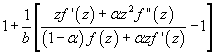(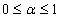and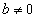be a complex number) lies in a region starlike with respect to 1 and is symmetric with respect to the real axis. Also certain applications of the main result for a class of functions of complex order defined by convolution are given. As a special case of this result, coefficient inequality for a class of functions defined through fractional derivatives is obtained. The motivation of this paper is to give a generalization of the coefficient inequalities of the subclasses of starlike and convex functions of complex order. 2: Paper Source PDF document Paper's Title: On Interaction of Discontinuous Waves in a Gas with Dust Particles Author(s): J. Jena Department of Mathematics, Netaji Subhas institute of technology, Sector-3, Dwarka, New Delhi - 110 075, India. jjena67@rediffmail.com jjena@nsit.ac.in Abstract: In this paper, the interaction of the strong shock with the weak discontinuity has been investigated for the system of partial differential equations describing one dimensional unsteady plane flow of an inviscid gas with large number of dust particles. The amplitudes of the reflected and transmitted waves after interaction of the weak discontinuity through a strong shock are evaluated by exploiting the results of general theory of wave interaction. 2: Paper Source PDF document Paper's Title: Local and Global Existence and Uniqueness Results for Second and Higher Order Impulsive Functional Differential Equations with Infinite Delay Author(s): Johnny Henderson and Abdelghani Ouahab Department of Mathematics, Baylor University, Waco, Texas 76798-7328 USA. Johnny_Henderson@baylor.edu Laboratoire de Mathématiques, Université de Sidi Bel Abbés BP 89, 22000 Sidi Bel Abbées, Algérie. ouahab@univ-sba.dz Abstract: In this paper, we discuss the local and global existence and uniqueness results for second and higher order impulsive functional differential equations with infinite delay. We shall rely on a nonlinear alternative of Leray-Schauder. For the global existence and uniqueness we apply a recent Frigon and Granas nonlinear alternative of Leray-Schauder type in Fréchet spaces. 2: Paper Source PDF document Paper's Title: An Approximation of Jordan Decomposable Functions for a Lipschitz Function Author(s): Ibraheem Alolyan Mathematics Department, College of Science, King Saud University P.O.Box: 2455, Riyadh 11451, Saudi Arabia ialolyan05@yahoo.com Abstract: The well known Jordan decomposition theorem gives the useful characterization that any function of bounded variation can be written as the difference of two increasing functions. Functions which can be expressed in this way can be used to formulate an exclusion test for the recent Cellular Exclusion Algorithms for numerically computing all zero points or the global minima of functions in a given cellular domain [2,8,9]. In this paper we give an algorithm to approximate such increasing functions when only the values of the function of bounded variation can be computed. For this purpose, we are led to introduce the idea of ε-increasing functions. It is shown that for any Lipschitz continuous function, we can find two ε-increasing functions such that the Lipschitz function can be written as the difference of these functions. 2: Paper Source PDF document Paper's Title: Contact With Adhesion between a Deformable Body and a Foundation Author(s): B. Teniou and M. Sofonea Laboratoire de Mathematiques Appliquées et Modélisation, Université Mentouri, Constantine 25000, Algeria tenioubou2@yahoo.fr Laboratoire de Mathématiques et Physiques pour les Systémes, Univesité de Perpignan, France. sofonea@univ-perp.fr Abstract: The aim of this work is study a dynamic contact problem between a deformable body and a foundation where the deformations are supposed to be small. The contact is with adhesion and normal compliance. The behavior of this body is modeled by a nonlinear elastic-visco-plastic law. The evolution of bonding field is described by a nonlinear differential equation. We derive a variational formulation of the contact problem and we prove the existence and uniqueness of its solution. The proof is based on the construction of three intermediate problems and then we construct a contraction mapping whose unique fixed point will be the weak solution of the mechanical problem. 2: Paper Source PDF document Paper's Title: Fixed Points and Stability of the Cauchy Functional Equation Author(s): Choonkil Park and Themistocles M. Rassias Department of Mathematics, Hanyang University, Seoul 133-791, Republic of Korea Department of Mathematics, National Technical University of Athens, Zografou Campus, 15780 Athens, Greece baak@hanyang.ac.kr trassias@math.ntua.gr Abstract: Using fixed point methods, we prove the generalized Hyers-Ulam stability of homomorphisms in Banach algebras and of derivations on Banach algebras for the Cauchy functional equation. 2: Paper Source PDF document Paper's Title: Fractional Integral Operators and Olsen Inequalities on Non-Homogeneous Spaces Author(s): Idha Sihwaningrum, Herry P. Suryawan, Hendra Gunawan Analysis and Geometry Group, Faculty of Mathematics and Natural Sciences, Bandung Institute of Technology, Bandung 40132, Indonesia hgunawan@math.itb.ac.id URL: http://personal.fmipa.itb.ac.id/hgunawan/ Abstract: We prove the boundedness of the fractional integral operator Iα on generalized Morrey spaces of non-homogeneous type. In addition, we also present Olsen-type inequalities for a multiplication operator involving Iα. Our proof uses a result of García-Cuerva and Martell . 2: Paper Source PDF document Paper's Title: A Generalization of a Trace Inequality for Positive Definite Matrices Author(s): E. V. Belmega, M. Jungers, and S. Lasaulce Université Paris-Sud Xi, SUPELEC, Laboratoire Des Signaux Et Systčmes, Gif-Sur-Yvette, France. belmega@lss.supelec.fr http://veronica.belmega.lss.supelec.fr CNRS, ENSEM, CRAN, Vandoeuvre, France. marc.jungers@cran.uhp-nancy.fr http://perso.ensem.inpl-nancy.fr/Marc.Jungers/ CNRS, SUPELEC, Laboratoire des Signaux et Systčmes, Gif-Sur-Yvette, France. lasaulce@lss.supelec.fr http://samson.lasaulce.lss.supelec.fr Abstract: In this note, we provide a generalization of the trace inequality derived in [Belmega].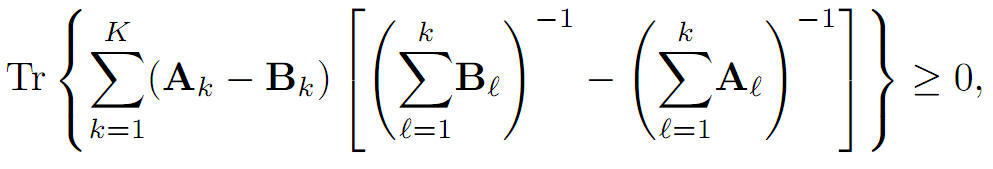More precisely, we prove that for arbitrary K ≥ 1 where Tr(∙) denotes the matrix trace operator, A1, B1 are any positive definite matrices and Ak, Bk for all k∈{2,...,k}, are any positive semidefinite matrices. 2: Paper Source PDF document Paper's Title: Uniform Convergence of Schwarz Method for Noncoercive Variational Inequalities Simple Proof Author(s): M. Haiour and E. Hadidi. Department of mathematics, LANOS Laboratory, Faculty of the Sciences, University Badji Mokhtar, P.O 23000 Annaba, Algeria. haiourm@yahoo.fr, ehadidi71@yahoo.fr Abstract: In this paper we study noncoercive variational inequalities, using the Schwarz method. The main idea of this method consists in decomposing the domain in two subdomains. We give a simple proof for the main result concerning L error estimates, using the Zhou geometrical convergence and the L approximation given for finite element methods by Courty-Dumont. 2: Paper Source PDF document Paper's Title: Approximation of an AQCQ-Functional Equation and its Applications Author(s): Choonkil Park and Jung Rye Lee Department of Mathematics, Research Institute for Natural Sciences, Hanyang University, Seoul 133-791, Korea; Department of Mathematics, Daejin University, Kyeonggi 487-711, Korea baak@hanyang.ac.kr jrlee@daejin.ac.kr Abstract: This paper is a survey on the generalized Hyers-Ulam stability of an AQCQ-functional equation in several spaces. Its content is divided into the following sections: 1. Introduction and preliminaries. 2. Generalized Hyers-Ulam stability of an AQCQ-functional equation in Banach spaces: direct method. 3. Generalized Hyers-Ulam stability of an AQCQ-functional equation in Banach spaces: fixed point method. 4. Generalized Hyers-Ulam stability of an AQCQ-functional equation in random Banach spaces: direct method. 5. Generalized Hyers-Ulam stability of an AQCQ-functional equation in random Banach spaces: fixed point method. 6. Generalized Hyers-Ulam stability of an AQCQ-functional equation in non-Archi-medean Banach spaces: direct method. 7. Generalized Hyers-Ulam stability of an AQCQ-functional equation in non-Archi-medean Banach spaces: fixed point method. 2: Paper Source PDF document Paper's Title: Growth and Products of Subharmonic Functions in the Unit Ball Author(s): R. Supper Université de Strasbourg, UFR de Mathématique et Informatique, URA CNRS 001, 7 rue René Descartes, F--67 084 Strasbourg Cedex, France raphaele.supper@math.unistra.fr Abstract: The purpose of this paper is to link information on the application u→gu with some growth conditions on the functions u and g subharmonic in the unit ball of RN. Two kinds of growth are considered: the Bloch--type growth and growth conditions expressed through integrals involving involutions of the unit ball. 2: Paper Source PDF document Paper's Title: Existence Results for Second Order Impulsive Functional Differential Equations with Infinite Delay Author(s): M. Lakrib, A. Oumansour and K. Yadi Laboratoire de Mathématiques, Université Djillali Liabées, B.P. 89 Sidi Bel Abbčs 22000, Algérie mlakrib@univ-sba.dz oumansour@univ-sba.dz Laboratoire de Mathématiques, Université Abou Bekr Belkaid, B.P. 119 Tlemcen 13000, Algérie k_yadi@mail.univ-tlemcen.dz Abstract: In this paper we study the existence of solutions for second order impulsive functional differential equations with infinite delay. To obtain our results, we apply fixed point methods. 2: Paper Source PDF document Paper's Title: A Geometric Generalization of Busemann-Petty Problem Author(s): Liu Rong and Yuan Jun Shanghai Zhangjiang Group Junior Middle School, Huo Xiang Road, Shanghai, 201203, China Abstract: The norm defined by Busemann's inequality establishes a class of star body - intersection body. This class of star body plays a key role in the solution of Busemann-Petty problem. In 2003, Giannapoulos  defined a norm for a new class of half-section. Based on this norm, we give a geometric generalization of Busemann-Petty problem, and get its answer as a result 2: Paper Source PDF document Paper's Title: New Inequalities of Mill's Ratio and Application to The Inverse Q-function Approximation Author(s): Pingyi Fan Department of Electronic Engineering, Tsinghua University, Beijing, China fpy@tsinghua.edu.cn Abstract: In this paper, we investigate the Mill's ratio estimation problem and get two new inequalities. Compared to the well known results obtained by Gordon, they becomes tighter. Furthermore, we also discuss the inverse Q-function approximation problem and present some useful results on the inverse solution. Numerical results confirm the validness of our theoretical analysis. In addition, we also present a conjecture on the bounds of inverse solution on Q-function. 2: Paper Source PDF document Paper's Title: A Generalization of Viete's Infinite Product and New Mean Iterations Author(s): Ryo Nishimura Department of Frontier Materials Nagoya Institute of Technology Gokiso-cho, Showa-ku, Nagoya Aichi, 466-8555, Japan. E-mail: rrnishimura@gmail.com Abstract: In this paper, we generalize Viéte's infinite product formula by use of Chebyshev polynomials. Furthermore, the infinite product formula for the lemniscate sine is also generalized. Finally, we obtain new mean iterations by use of these infinite product formulas. 2: Paper Source PDF document Paper's Title: Applications of the Structure Theorem of (w1,w2)-Tempered Ultradistributions Author(s): Hamed M. Obiedat and Lloyd E. Moyo Department of Mathematics, Hashemite University, P.O.Box 150459, Zarqa13115, Jordan. E-mail: hobiedat@hu.edu.j Department of Mathematics, Computer Science & Statistics, Henderson State University, 1100 Henderson Street, Arkadelphia, AR 71999, USA. E-mail: moyol@hsu.edu Abstract: Using a previously obtained structure theorem for (w1, w2)-tempered ultradistributions, we prove that these ultradistributions can be represented as initial values of solutions of the heat equation. 2: Paper Source PDF document Paper's Title: MSplit Equality for Monotone Inclusion Problem and Fixed Point Problem in Real Banach Spaces Author(s): 1,2Christian Chibueze Okeke, 3Abdumalik Usman Bello, 1Chinedu Izuchukwu, and 1Oluwatosin Temitope Mewomo 1School of Mathematics, Statistics and Computer Science, University of KwaZulu-Natal, Durban, South Africa. E-mail: okekec@ukzn.ac.za E-mail: izuchukwuc@ukzn.ac.za E-mail: mewomoo@ukzn.ac.za 2DST-NRF Center of Excellence in Mathematical and Statistical Sciences (CoE-Mass) Johannesburg, South Africa. 3Federal University, Dutsin-Ma, Katsina State, Nigeria. E-mail: uabdulmalik@fudutsinma.edu.ng Abstract: In this paper a new iterative algorithm for approximating a common solution of split equality monotone inclusion problem and split equality fixed point problem is introduced. Using our algorithm, we state and prove a strong convergence theorem for approximating an element in the intersection of the set of solutions of a split equality monotone inclusion problem and the set of solutions of a split equality fixed point problem for right Bregman strongly nonexpansive mappings in the setting of p-uniformly convex Banach spaces which are also uniformly smooth. We also give some applications. 2: Paper Source PDF document Paper's Title: Inequalities for Discrete F-Divergence Measures: A Survey of Recent Results Author(s): Sever S. Dragomir1,2 1Mathematics, School of Engineering & Science Victoria University, PO Box 14428 Melbourne City, MC 8001, Australia E-mail: sever.dragomir@vu.edu.au 2DST-NRF Centre of Excellence in the Mathematical and Statistical Sciences, School of Computer Science & Applied Mathematics, University of the Witwatersrand, Private Bag 3, Johannesburg 2050, South Africa URL: http://rgmia.org/dragomir Abstract: In this paper we survey some recent results obtained by the author in providing various bounds for the celebrated f-divergence measure for various classes of functions f. Several techniques including inequalities of Jensen and Slater types for convex functions are employed. Bounds in terms of Kullback-Leibler Distance, Hellinger Discrimination and Varation distance are provided. Approximations of the f-divergence measure by the use of the celebrated Ostrowski and Trapezoid inequalities are obtained. More accurate approximation formulae that make use of Taylor's expansion with integral remainder are also surveyed. A comprehensive list of recent papers by several authors related this important concept in information theory is also included as an appendix to the main text. 2: Paper Source PDF document Paper's Title: Birkhoff-James orthogonality and Best Approximant in L1(X) Author(s): Mecheri Hacene and Rebiai Belgacem Department of Mathematics and Informatics, LAMIS laboratory, University of Tebessa, Algeria. E-mail: mecherih2000@yahoo.fr Department of Mathematics and Informatics, LAMIS laboratory, University of Tebessa, Algeria. E-mail: brebiai@gmail.com Abstract: Let X be a complex Banach space and let (X,ρ) be a positive measure space. The Birkhoff-James orthogonality is a generalization of Hilbert space orthogonality to Banach spaces. We use this notion of orthogonality to establish a new characterization of Birkhoff-James orthogonality of bounded linear operators in L1(X,ρ) also implies best approximation has been proved. 2: Paper Source PDF document Paper's Title: Polyanalytic Functions on Subsets of Z[i] Author(s): Abtin Daghighi Linköping University, SE-581 83, Sweden. E-mail: abtindaghighi@gmail.com Abstract: For positive integers q we consider the kernel of the powers Lq where L is one of three kinds of discrete analogues of the Cauchy-Riemann operator. The first two kinds are well-studied, but the third kind less so. We give motivations for further study of the third kind especially since its symmetry makes it more appealing for the cases q 2. From an algebraic perspective it makes sense that the chosen multiplication on the kernels is compatible with the choice of pseudo-powers. We propose such multiplications together with associated pseudo-powers. We develop a proof-tool in terms of certain sets of uniqueness. 2: Paper Source PDF document Paper's Title: A Method of the Study of the Cauchy Problem for~a~Singularly Perturbed Linear Inhomogeneous Differential Equation Author(s): E. E. Bukzhalev and A. V. Ovchinnikov Faculty of Physics, Moscow State University, 1 Leninskie Gory, Moscow, 119991, Russia E-mail: bukzhalev@mail.ru Russian Institute for Scientific and Technical Information of the Russian Academy of Sciences, 20 Usievicha St., Moscow, 125190, Russia E-mail: ovchinnikov@viniti.ru Abstract: We construct a sequence that converges to a solution of the Cauchy problem for a singularly perturbed linear inhomogeneous differential equation of an arbitrary order. This sequence is also an asymptotic sequence in the following sense: the deviation (in the norm of the space of continuous functions) of its nth element from the solution of the problem is proportional to the (n+1)th power of the parameter of perturbation. This sequence can be used for justification of asymptotics obtained by the method of boundary functions. 2: Paper Source PDF document Paper's Title: The Influence of Fluid Pressure in Macromechanical Cochlear Model Author(s): F. E. Aboulkhouatem1, F. Kouilily1, N. Achtaich1, N. Yousfi1 and M. El Khasmi2 1Department of Mathematics and Computer Science, Faculty of Sciences Ben M'sik, Hassan II University, Casablanca, Morocco. 2Department of Biology, Faculty of Sciences Ben M'sik, Hassan II University, Casablanca, Morocco. E-mail: fatiaboulkhouatem@gemail.com URL: http://www.fsb.univh2c.ma/ Abstract: An increase of pressure in the structure of cochlea may cause a hearing loss. In this paper, we established the relationship between the fluid pressure and the amplitude of displacement of Basilar Membrane to clarify the mechanisms of hearing loss caused by increasing of this pressure. So, a mathematical cochlear model was formulated using finite difference method in order to explain and demonstrate this malfunction in passive model. Numerical simulations may be considered as helpful tools which may extend and complete the understanding of a cochlea dysfunction. 2: Paper Source PDF document Paper's Title: Dynamical Analysis of HIV/AIDS Epidemic Model with Two Latent Stages, Vertical Transmission and Treatment Author(s): Nur Shofianah, Isnani Darti, Syaiful Anam Mathematics Department,Faculty of Mathematics and Natural Sciences. University of Brawijaya, Jl. Veteran, Malang 65145, Indonesia. E-mail: nur_shofianah@ub.ac.id, isnanidarti@ub.ac.id, syaiful@ub.ac.id Abstract: We discuss about dynamical analysis of HIV/AIDS epidemic model with two latent stages, vertical transmission and treatment. In this model, the spreading of HIV occurs through both horizontal and vertical transmission. There is also treatment for individual who has been HIV infected. The latent stage is divided into slow and fast latent stage based on the immune condition which varies for each individual. Dynamical analysis result shows that the model has two equilibrium points: the disease-free equilibrium point and the endemic equilibrium point. The existence and global stability of equilibrium points depend on the basic reproduction number R0. When R0 <1, only the disease-free equilibrium point exists. If R0 >1, there are two equilibrium points, which are the disease-free equilibrium point and the endemic equilibrium point. Based on the result of stability analysis, the disease-free equilibrium point is globally asymptotically stable if R0 <1, while if R0 > 1 and p=q, the endemic equilibrium point will be globally asymptotically stable. In the end, we show some numerical simulations to support the analytical result. 2: Paper Source PDF document Paper's Title: Weyl's theorem for class Q and k - quasi class Q Operators Author(s): S. Parvatham and D. Senthilkumar Department of Mathematics and Humanities, Sri Ramakrishna Institute of Technology, Coimbatore-10, Tamilnadu, India. E-mail: parvathasathish@gmail.com Post Graduate and Research Department of Mathematics, Govt. Arts College, Coimbatore-641018, Tamilnadu, India. E-mail: senthilsenkumhari@gmail.com Abstract: In this paper, we give some properties of class Q operators. It is proved that every class Q operators satisfies Weyl's theorem under the condition that T2 is isometry. Also we proved that every k quasi class Q operators is Polaroid and the spectral mapping theorem holds for this class of operator. It will be proved that single valued extension property, Weyl and generalized Weyl's theorem holds for every k quasi class Q operators. 2: Paper Source PDF document Paper's Title: Analysis of a Dynamic Elasto-viscoplastic Frictionless Antiplan Contact Problem with Normal Compliance Author(s): A. Ourahmoun1, B. Bouderah2, T. Serrar3 1,2Applied Mathematics Laboratory, M'sila University, 28000, Algeria. E-mail: ourahmounabbes@yahoo.fr 3Applied Mathematics Laboratory, Setif 1 University, 19000, Algeria. Abstract: We consider a mathematical model which describes the dynamic evolution of a thermo elasto viscoplastic contact problem between a body and a rigid foundation. The mechanical and thermal properties of the obstacle coating material near its surface. A variational formulation of this dynamic contact phenomenon is derived in the context of general models of thermo elasto viscoplastic materials. The displacements and temperatures of the bodies in contact are governed by the coupled system consisting of a variational inequality and a parabolic differential equation. The proof is based on a classical existence and uniqueness result on parabolic inequalities,differential equations and fixed point arguments. 2: Paper Source PDF document Paper's Title: Coexisting Attractors and Bubbling Route to Chaos in Modified Coupled Duffing Oscillators Author(s): B. Deruni1, A. S. Hacinliyan1,2, E. Kandiran3, A. C. Keles2, S. Kaouache4, M.-S. Abdelouahab4, N.-E. Hamri4 1Department of Physics, University of Yeditepe, Turkey. 2Department of Information Systems and Technologies, University of Yeditepe, Turkey 3Department of Software Development, University of Yeditepe, Turkey. 4Laboratory of Mathematics and their interactions, University Center of Abdelhafid Boussouf, Mila 43000, Algeria. E-mail: berc890@gmail.com ahacinliyan@yeditepe.edu.tr engin.kandiran@yeditepe.edu.tr cihan.keles@yeditepe.edu.tr s.kaouache@centr-univ-mila.dz medsalah3@yahoo.fr n.hamri@centre-univ-mila.dz Abstract: In this article dynamical behavior of coupled Duffing oscillators is analyzed under a small modification. The oscillators have cubic damping instead of linear one. Although single duffing oscillator has complex dynamics, coupled duffing systems possess a much more complex structure. The dynamical behavior of the system is investigated both numerically and analytically. Numerical results indicate that the system has double scroll attractor with suitable parameter values. On the other hand, bifurcation diagrams illustrate rich behavior of the system, and it is seen that, system enters into chaos with different routes. Beside classical bifurcations, bubbling route to chaos is observed for suitable parameter settings. On the other hand, Multistability of the system is indicated with the coexisting attractors, such that under same parameter setting the system shows different periodic and chaotic attractors. Moreover, chaotic synchronization of coupled oscillators is illustrated in final section. 1: Paper Source PDF document Paper's Title: Pseudomonotonicity and Quasimonotonicity by Translations versus Monotonicity in Hilbert Spaces Author(s): George Isac and Dumitru Motreanu Department of Mathematics, Royal Military College of Canada, P.O. Box 17000 Stn Forces Kingston, Ontario, Canada, K7k 7b4. Département de Mathématiques, Université de Perpignan, 66860 Perpignan, France. Abstract: Let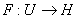be a Gâteaux differentiable mapping on an open convex subset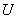of a Hilbert space. If there exists a straight line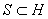such that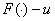is pseudomonotone for any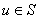then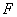is monotone. Related results using a regularity condition are given. 1: Paper Source PDF document Paper's Title: Long Time Behavior for a Viscoelastic Problem with a Positive Definite Kernel Author(s): Nasser-eddine Tatar King Fahd University of Petroleum and Mineral, Department of Mathematical Sciences, Dhahran, 31261 Saudi Arabia Abstract: We study the asymptotic behavior of solutions for an integro-differential problem which arises in the theory of viscoelasticity. It is proved that solutions go to rest in an exponential manner under new assumptions on the relaxation function in the memory term. In particular, we consider a new family of kernels which are not necessarily decreasing. 1: Paper Source PDF document Paper's Title: On Sufficient Conditions for Strong Starlikeness Author(s): V. Ravichandran, M. H. Khan, M. Darus, And K. G. Subramanian School of Mathematical Sciences, Universiti Sains Malaysia, 11800 Usm Penang, Malaysia Url : h ttp://cs.usm.my/~vravi/index.html Department of Mathematics, Islamiah College, Vaniambadi 635 751, India khanhussaff@yahoo.co.in School of Mathematical Sciences, Faculty of Science and Technology, UKM, Bangi 43600, M alaysia maslina@pkrisc.cc.ukm.my Url : http://www.webspawner.com/users/maslinadarus Department of Mathematics, Madras Christian College, Tambaram, Chennai 600 059, India Abstract: In the present investigation, we obtain some sufficient conditions for a normalized analytic function f(z) defined on the unit disk to satisfy the condition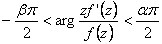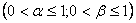1: Paper Source PDF document Paper's Title: Fekete-Szegö Inequality for Certain Class of Analytic Functions Author(s): V. Ravichandran, Maslina Darus, M. Hussain Khan, and K. G. Subramanian School of Mathematical Sciences, Universiti Sains Malaysia, 11800 Usm, Penang, Malaysia School of Mathematical Sciences, Faculty of Sciences and Technology, Ukm, Banki 43600, Malaysia Department of Mathematics, Islamiah College, V aniambadi 635 751, India Department of Mathematics, Madras Christian College, Tambaram, Chennai- 600 059, India Abstract: In this present investigation, the authors obtain Fekete-Szegö inequality for a certain class of analytic functions f(z) for which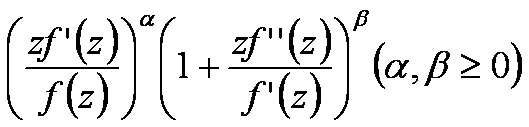lies in a region starlike with respect to 1 and symmetric with respect to the real axis. Also certain application of our main result for a class of functions defined by Hadamard product (convolution) is given. As a special case of our result we obtain Fekete-Szegö inequality for a class of functions defined through fractional derivatives. Also we obtain Fekete-Szegö inequality for the inverse functions. 1: Paper Source PDF document Paper's Title: The successive approximations method and error estimation in terms of at most the first derivative for delay ordinary differential equations Author(s): Alexandru Mihai Bica Department of Mathematics, University of Oradea, Str. Armatei Romane no.5, 410087, Oradea, Romania smbica@yahoo.com abica@uoradea.ro Abstract: We present here a numerical method for first order delay ordinary differential equations, which use the Banach's fixed point theorem, the sequence of successive approximations and the trapezoidal quadrature rule. The error estimation of the method uses a recent result of P. Cerone and S.S. Dragomir about the remainder of the trapezoidal quadrature rule for Lipchitzian functions and for functions with continuous first derivative. 1: Paper Source PDF document Paper's Title: An Easy and Efficient Way for Solving A class of Singular Two Point Boundary Value Problems Author(s): Muhammed I. Syam, Muhammed N. Anwar and Basem S. Attili Mathematical Sciences Department United Arab Emirates University, P. O. Box 17551 Al-Ain, United Arab Emirates b.attili@uaeu.ac.ae Abstract: We will consider an efficient and easy way for solving a certain class of singular two point boundary value problems. We will employ the least squares method which proved to be efficient for this type of problems. Enough examples that were considered by others will be solved with comparison with the results presented there. 1: Paper Source PDF document Paper's Title: Comparison Results for Solutions of Time Scale Matrix Riccati Equations and Inequalities Author(s): R. Hilscher Department of Mathematical Analysis, Faculty of Science, Masaryk University, Jan áčkovo nám. 2a, CZ-60200, Brno, Czech Republic. hilscher@math.muni.cz URL: http://www.math.muni.cz/~hilscher/ Abstract: In this paper we derive comparison results for Hermitian solutions of time scale matrix Riccati equations and Riccati inequalities. Such solutions arise from special conjoined bases (X,U) of the corresponding time scale symplectic system via the Riccati quotient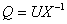. We also discuss properties of a unitary matrix solution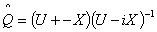of a certain associated Riccati equation. 1: Paper Source PDF document Paper's Title: Iterated Order of Fast Growth Solutions of Linear Differential Equations Author(s): Benharrat Belaďdi Department of Mathematics Laboratory of Pure and Applied Mathematics University of Mostaganem B. P. 227 Mostaganem, ALGERIA. belaidi@univ-mosta.dz Abstract: In this paper, we investigate the growth of solutions of the differential equation f(k) + Ak-1 (z) f(k-1) +...+ A1 (z) f' + A0 (z) f= F (z), where Ao (z), ..., Ak-1 (z) and F (z)0 are entire functions. Some estimates are given for the iterated order of solutions of the above quation when one of the coefficients As is being dominant in the sense that it has larger growth than Aj (j≠s) and F. 1: Paper Source PDF document Paper's Title: Normalized Truncated Levy models applied to the study of Financial Markets Author(s): M. C. Mariani, K. Martin, D. W. Dombrowski and D. Martinez Department of Mathematical Sciences and Department of Finance, New Mexico State University, P.O. Box 30001 Department 3MB Las Cruces, New Mexico 88003-8001 USA. mmariani@nmsu.edu kjmartin@nmsu.edu Abstract: This work is devoted to the study of the statistical properties of financial instruments from developed markets. We performed a new analysis of the behavior of companies corresponding to the DJIA index, and of the index itself, by using a normalized Truncated Levy walk model. We conclude that the Truncated Levy distribution describes perfectly the evolution of the companies and of the index near a crash. 1: Paper Source PDF document Paper's Title: Existence of Bounded Solutions for a Class of Strongly Nonlinear Elliptic Equations in Orlicz-Sobolev Spaces Author(s): Abdelmoujib Benkirane and Ahmed Youssfi Department of Mathematics and Informatics, Faculty of Sciences Dhar El Mahraz University Sidi Mohammed Ben Abdallah PB 1796 Fez-Atlas, Fez Morocco a.benkirane@menara.ma ahmed.youssfi@caramail.com Abstract: We prove, in the setting of Orlicz-Sobolev spaces, the existence of bounded solutions for some strongly nonlinear elliptic equations with operator of the principal part having degenerate coercivity and lower order terms not satisfying the sign condition. The data have a suitable summability and no Δ2-condition is needed for the considered N-functions. 1: Paper Source PDF document Paper's Title: On Some Remarkable Product of Theta-function Author(s): M. S. Mahadeva Naika, M. C. Maheshkumar and K. Sushan Bairy Department of Mathematics, Bangalore University, Central College Campus, Bangalore-560 001, INDIA msmnaika@rediffmail.com softmahe@rediffmail.com ksbairy@gmail.com Abstract: On pages 338 and 339 in his first notebook, Ramanujan records eighteen values for a certain product of theta-function. All these have been proved by B. C. Berndt, H. H. Chan and L-C. Zhang . Recently M. S. Mahadeva Naika and B. N. Dharmendra [7, 8] and Mahadeva Naika and M. C. Maheshkumar  have obtained general theorems to establish explicit evaluations of Ramanujan's remarkable product of theta-function. Following Ramanujan we define a new function bM,N as defined in (1.5). The main purpose of this paper is to establish some new general theorems for explicit evaluations of product of theta-function. 1: Paper Source PDF document Paper's Title: The Bernoulli Inequality in Uniformly Complete f-algebras with Identity Author(s): Adel Toumi and Mohamed Ali Toumi Laboratoire de Physique des Liquides Critiques, Département de Physique, Faculté des Sciences de Bizerte, 7021, Zarzouna, Bizerte, TUNISIA Adel.Toumi@fst.rnu.tn Département de Mathématiques, Faculté des Sciences de Bizerte, 7021, Zarzouna, Bizerte, TUNISIA MohamedAli.Toumi@fsb.rnu.tn Abstract: The main purpose of this paper is to establish with a constructive proof the Bernoulli inequality: let A be a uniformly complete f-algebra with e as unit element, let 1<p<∞, then (e+a)p≥e+pa for all a∈A+. As an application we prove the Hölder inequality for positive linear functionals on a uniformly complete f-algebra with identity by using the Minkowski inequality. 1: Paper Source PDF document Paper's Title: Existence Results for Perturbed Fractional Differential Inclusions Author(s): Y.-K. Chang Department of Mathematics, Lanzhou Jiaotong University, Lanzhou, Gansu 730070, People's Republic of China lzchangyk@163.com Abstract: This paper is mainly concerned with the following fractional differential inclusions with boundary condition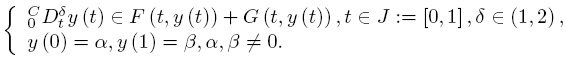A sufficient condition is established for the existence of solutions of the above problem by using a fixed point theorem for multivalued maps due to Dhage. Our result is proved under the mixed generalized Lipschitz and Carathéodory conditions. 1: Paper Source PDF document Paper's Title: A Fixed Point Approach to the Stability of the Equation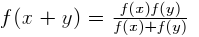Author(s): Soon-Mo Jung Mathematics Section, College of Science and Technology Hong-Ik University, 339-701 Chochiwon Republic of Korea. smjung@hongik.ac.kr Abstract: We will apply a fixed point method for proving the Hyers--Ulam stability of the functional equation. 1: Paper Source PDF document Paper's Title: Ergodic Solenoidal Homology II: Density of Ergodic Solenoids Author(s): Vicente Muńoz and Ricardo Pérez Marco Instituto de Ciencias Matemáticas CSIC-UAM-UC3M-UCM, Serrano 113 bis, 28006 Madrid, Spain and Facultad de Matem áticas, Universidad Complutense de Madrid, Plaza de Ciencias 3, 28040 Madrid, Spain CNRS, LAGA UMR 7539, Universit é Paris XIII, 99 Avenue J.-B. Cl\'ement, 93430-Villetaneuse, France vicente.munoz@imaff.cfmac.csic.es ricardo@math.univ-paris13.fr Abstract: A measured solenoid is a laminated space endowed with a tranversal measure invariant by holonomy. A measured solenoid immersed in a smooth manifold produces a closed current (known as a generalized Ruelle-Sullivan current). Uniquely ergodic solenoids are those for which there is a unique (up to scalars) transversal measure. It is known that for any smooth manifold, any real homology class is represented by a uniquely ergodic solenoid. In this paper, we prove that the currents associated to uniquely ergodic solenoids are dense in the space of closed currents, therefore proving the abundance of such objects. 1: Paper Source PDF document Paper's Title: A Study of the Effect of Density Dependence in a Matrix Population Model Author(s): N. Carter and M. Predescu Department of Mathematical Sciences, Bentley University, Waltham, MA 02452, U.S.A. ncarter@bentley.edu mpredescu@bentley.edu Abstract: We study the behavior of solutions of a three dimensional discrete time nonlinear matrix population model. We prove results concerning the existence of equilibrium points, boundedness, permanence of solutions, and global stability in special cases of interest. Moreover, numerical simulations are used to compare the dynamics of two main forms of the density dependence function (rational and exponential). 1: Paper Source PDF document Paper's Title: A Coincidence Theorem for Two Kakutani Maps Author(s): Mircea Balaj Department of Mathematics, University of Oradea, 410087, Oradea, Romania. mbalaj@uoradea.ro Abstract: In this paper we prove the following theorem: Let X be a nonempty compact convex set in a locally convex Hausdorff topological vector space, D be the set of its extremal points and F,T: XX two Kakutani maps; if for each nonempty finite subset A of D and for any x ∈ coA, F (x) coA ≠ Ř, then F and T have a coincidence point. The proof of this theorem is given first in the case when X is a simplex, then when X is a polytope and finally in the general case. Several reformulations of this result are given in the last part of the paper. 1: Paper Source PDF document Paper's Title: Real Interpolation Methods and Quasilogarithmic Operators Author(s): Ming Fan School of Industrial Technology and Management, Dalarna University, 781 88 Borlänge, Sweden fmi@du.se URL: http://users.du.se/~fmi Abstract: The purpose of this paper is to deal with nonlinear quasilogarithmic operators, which possesses the uniformly bounded commutator property on various interpolation spaces in the sense of Brudnyi-Krugljak associated with the quasi-power parameter spaces. The duality, and the domain and range spaces of these operators are under consideration. Some known inequalities for the Lebesgue integration spaces and the trace classes are carried over to the non-commutative symmetric spaces of measurable operators affiliated with a semi-finite von Neumann algebra. 1: Paper Source PDF document Paper's Title: Shape Diagrams for 2D Compact Sets - Part III: Convexity Discrimination for Analytic and Discretized Simply Connected Sets. Author(s): S. Rivollier, J. Debayle and J.-C. Pinoli Ecole Nationale Supérieure des Mines de Saint-Etienne, CIS - LPMG, UMR CNRS 5148, 158 cours Fauriel, 42023 Saint-Etienne Cedex 2, France. Abstract: Shape diagrams are representations in the Euclidean plane introduced to study 3-dimensional and 2-dimensional compact convex sets. However, they can also been applied to more general compact sets than compact convex sets. A compact set is represented by a point within a shape diagram whose coordinates are morphometrical functionals defined as normalized ratios of geometrical functionals. Classically, the geometrical functionals are the area, the perimeter, the radii of the inscribed and circumscribed circles, and the minimum and maximum Feret diameters. They allow twenty-two shape diagrams to be built. Starting from these six classical geometrical functionals, a detailed comparative study has been performed in order to analyze the representation relevance and discrimination power of these twenty-two shape diagrams. The two first parts of this study are published in previous papers 8,9. They focus on analytic compact convex sets and analytic simply connected compact sets, respectively. The purpose of this paper is to present the third part, by focusing on the convexity discrimination for analytic and discretized simply connected compact sets.. 1: Paper Source PDF document Paper's Title: The Superstability of the Pexider Type Trigonometric Functional Equation Author(s): Gwang Hui Kim and Young Whan Lee Department of Mathematics, Kangnam University Yongin, Gyeonggi, 446-702, Korea. ghkim@kangnam.ac.kr Department of Computer and Information Security Daejeon University, Daejeon 300-716, Korea. ywlee@dju.ac.kr Abstract: The aim of this paper is to investigate the stability problem for the Pexider type (hyperbolic) trigonometric functional equation f(x+y)+f(x+σy)=λg(x)h(y) under the conditions : |f(x+y)+f(x+σy)- λg(x)h(y)|≤φ(x), φ(y) , and min {φ(x), φ (y)}. As a consequence, we have generalized the results of stability for the cosine(d'Alembert), sine, and the Wilson functional equations by J. Baker, P. Găvruta, R. Badora and R. Ger, Pl.~Kannappan, and G. H. Kim 1: Paper Source PDF document Paper's Title: Hyperbolic Models Arising in the Theory of Longitudinal Vibration of Elastic Bars Author(s): 1I. Fedotov, 1J. Marais, 1,2M. Shatalov and 1H.M. Tenkam 1Department of Mathematics and Statistics, Tshwane University of Technology Private Bag X6680, Pretoria 0001 South Africa. fedotovi@tut.ac.za, julian.marais@gmail.com, djouosseutenkamhm@tut.ac.za. 2Manufacturing and Materials Council of Scientific and Industrial Research (CSIR) P.O. Box 395, Pretoria, 0001 South Africa. mshatlov@csir.co.za Abstract: In this paper a unified approach to the derivation of families of one dimensional hyperbolic differential equations and boundary conditions describing the longitudinal vibration of elastic bars is outlined. The longitudinal and lateral displacements are expressed in the form of a power series expansion in the lateral coordinate. Equations of motion and boundary conditions are derived using Hamilton's variational principle. Most of the well known models in this field fall within the frames of the proposed theory, including the classical model, and the more elaborated models proposed by by Rayleigh, Love, Bishop, Mindlin, Herrmann and McNiven. The exact solution is presented for the Mindlin-Herrmann case in terms of Green functions. Finally, deductions regarding the accuracy of the models are made by comparison with the exact Pochhammer-Chree solution for an isotropic cylinder. 1: Paper Source PDF document Paper's Title: Some Inequalities for Gramian Normal Operators and for Gramian Self-Adjoint Operators in Pseudo-Hilbert Spaces Author(s): Loredana Ciurdariu Department of Mathematics,"Politehnica" University of Timisoara, P-ta. Victoriei, No.2, 300006-Timisoara, ROMANIA cloredana43@yahoo.com. Abstract: Several inequalities for gramian normal operators and for gramian self-adjoint operators in pseudo-Hilbert spaces are presented. 1: Paper Source PDF document Paper's Title: Hardy Type Inequalities via Convexity - The Journey so Far Author(s): James A. Oguntuase and Lars-Erik Persson Department of Mathematics, University of Agriculture, P. M. B. 2240, Abeokuta, Nigeria. Department of Mathematics, Luleĺ University of Technology, SE-971 87, Luleĺ , Sweden. oguntuase@yahoo.com, larserik@sm.luth.se . Abstract: It is nowadays well-known that Hardy's inequality (like many other inequalities) follows directly from Jensen's inequality. Most of the development of Hardy type inequalities has not used this simple fact, which obviously was unknown by Hardy himself and many others. Here we report on some results obtained in this way mostly after 2002 by mainly using this fundamental idea. 1: Paper Source PDF document Paper's Title: Generalizing Polyhedra to Infinite Dimension Author(s): Paolo d'Alessandro Department of Mathematics, Third University of Rome, Lgo S.L. Murialdo 1, 00146 Rome, Italy. dalex@mat.uniroma3.it. URL: http://www.mat.uniroma3.it/users/dalex/dalex.html. Abstract: This paper generalizes polyhedra to infinite dimensional Hilbert spaces as countable intersections of closed semispaces. Highlights are the structure theory that shows that a polyhedron is the sum of compact set (in a suitable topology) plus a closed pointed cone plus a closed subspace, giving the internal representation of polyhedra. In the final part the dual range space technique is extended to the solution of infinite dimensional LP problems. 1: Paper Source PDF document Paper's Title: A New Property of General Means of Order p with an Application to the Theory of Economic Growth Author(s): Olivier de La Grandville Department of Management Science and Engineering, Huang Engineering Center, Stanford University, 475 Via Ortega, Stanford, California 94305 U.S.A. lagrandvil@aol.com Abstract: The purpose of this note is to demonstrate a new property of the general mean of order p of m ordered positive numbers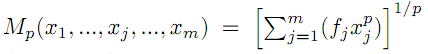. If p < 0 and if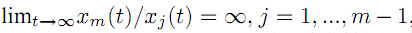, the elasticity of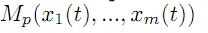with respect to xm, defined by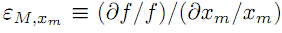, tends towards zero, and therefore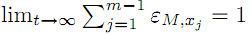. This property is then applied to optimal growth theory. 1: Paper Source PDF document Paper's Title: On the Inequality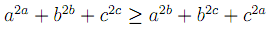Author(s): A. Coronel and F. Huancas Departamento de Ciencias Básicas, Facultad de Ciencias, Universidad del Bío-Bío, Casilla 447, Campus Fernando May, Chillán, Chile. acoronel@roble.fdo-may.ubiobio.cl Departamento Académico de Matemática, Facultad de Ciencias Físicas y Matemáticas, Universidad Nacional Pedro Ruiz Gallo, Juan XIII s/n, Lambayeque, Perú Abstract: In this paper we give a complete proof offor all positive real numbers a, b and c. Furthermore, we present another way to prove the statement for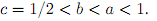1: Paper Source PDF document Paper's Title: Finite and Infinite Order Solutions of a Class of Higher Order Linear Differential Equations Author(s): Saada Hamouda Department of Mathematics, Laboratory of Pure and Applied Mathematics, University of Mostaganem, B. P 227 Mostaganem, ALGERIA hamouda_saada@yahoo.fr Abstract: In this paper, we investigate the growth of solutions of higher order linear differential equations where most of the coefficients have the same order and type with each other. 1: Paper Source PDF document Paper's Title: Equivalence of the Nonsmooth Nonlinear Complementarity Problems to Unconstrained Minimization Author(s): M. A. Tawhid and J. L. Goffin Department of Mathematics and Statistics, School of Advanced Technologies and Mathematics, Thompson Rivers University, 900 McGill Road, PO Box 3010, Kamloops, BC V2C 5N3 Canada Alexandria University and Egypt Japan University of Science and Technology, Alexandria-Egypt mtawhid@tru.ca Faculty of Management, McGill University, 1001 Sherbrooke Street West, Montreal, Quebec, H3A 1G5 Canada. Jean-Louis.Goffin@McGill.ca Abstract: This paper deals with nonsmooth nonlinear complementarity problem, where the underlying functions are nonsmooth which admit the H-differentiability but not necessarily locally Lipschitzian or directionally differentiable. We consider a reformulation of the nonlinear complementarity problem as an unconstrained minimization problem. We describe H-differentials of the associated penalized Fischer-Burmeister and Kanzow and Kleinmichel merit functions. We show how, under appropriate P0, semimonotone (E0), P, positive definite, and strictly semimonotone (E) -conditions on an H-differential of f, finding local/global minimum of a merit function (or a `stationary point' of a merit function) leads to a solution of the given nonlinear complementarity problem. Our results not only give new results but also unify/extend various similar results proved for C1. 1: Paper Source PDF document Paper's Title: A Note on Mixed Norm Spaces of Analytic Functions Author(s): K. Avetisyan Faculty of Physics, Yerevan State University, Alex Manoogian st. 1, Yerevan, 375025, Armenia avetkaren@ysu.am Abstract: A direct and elementary proof of an estimate of Littlewood is given together with an application concerning the sharpness and strictness of some inclusions in mixed norm spaces of analytic functions. 1: Paper Source PDF document Paper's Title: Some Distortion and Other Properties Associated with a Family of the n-Fold Symmetric Koebe Type Functions Author(s): H. M. Srivastava, N. Tuneski and E. Georgieva-Celakoska Department of Mathematics and Statistics, University of Victoria, Victoria, British Columbia V8W 3R4, Canada harimsri@math.uvic.ca Faculty of Mechanical Engineering, St. Cyril and Methodius University, Karpo'v s II b.b., MK-1000 Skopje, Republic of Macedonia Abstract: In a recent work by Kamali and Srivastava , a certain family of the n-fold symmetric Koebe type functions was introduced and studied systematically. In an earlier investigation, Eguchi and Owa  had considered its special case when n=1 (see also ). Here, in our present sequel to these earlier works, this general family of the n-fold symmetric Koebe type functions is studied further and several distortion theorems and such other properties as the radii of spirallikeness, the radii of starlikeness and the radii of convexity, which are associated with this family of the n-fold symmetric Koebe type functions, are obtained. We also provide certain criteria that embed this family of the n-fold symmetric Koebe type functions in a function class Gλ which was introduced and studied earlier by Silverman . 1: Paper Source PDF document Paper's Title: Szegö Limits and Haar Wavelet Basis Author(s): M. N. N. Namboodiri and S. Remadevi Dept. of Mathematics, Cochin University of Science and Technology, Cochin-21, Kerala, India. nambu@cusat.ac.in Dept. of Mathematics, College of Engineering, Cherthala, Kerala, India. rema@mec.ac.in Abstract: This paper deals with Szegö type limits for multiplication operators on L2 (R) with respect to Haar orthonormal basis. Similar studies have been carried out by Morrison for multiplication operators Tf using Walsh System and Legendre polynomials . Unlike the Walsh and Fourier basis functions, the Haar basis functions are local in nature. It is observed that Szegö type limit exist for a class of multiplication operators Tf , f∈ L (R) with respect to Haar (wavelet) system with appropriate ordering. More general classes of orderings of Haar system are identified for which the Szegö type limit exist for certain classes of multiplication operators. Some illustrative examples are also provided. 1: Paper Source PDF document Paper's Title: Positive Solutions to a System of Boundary Value Problems for Higher-Dimensional Dynamic Equations on Time Scales Author(s): I. Y. Karaca Department of Mathematics, Ege University, 35100 Bornova, Izmir, Turkey ilkay.karaca@ege.edu.tr URL: http://ege.edu.tr Abstract: In this paper, we consider the system of boundary value problems for higher-dimensional dynamic equations on time scales. We establish criteria for the existence of at least one or two positive solutions. We shall also obtain criteria which lead to nonexistence of positive solutions. Examples applying our results are also given. 1: Paper Source PDF document Paper's Title: A One-Line Derivation of the Euler and Ostrogradski Equations Author(s): Olivier de La Grandville Stanford University, Department of Management Science and Engineering, Stanford, CA 94305, U. S. A Abstract: At the very heart of major results of classical physics, the Euler and Ostrogradski equations have apparently no intuitive interpretation. In this paper we show that this is not so. Relying on Euler's initial geometric approach, we show that they can be obtained through a direct reasoning that does not imply any calculation. The intuitive approach we suggest offers two benefits: it gives immediate significance to these fundamental second-order non-linear differential equations; and second, it allows to obtain a property of the calculus of variations that does not seem to have been uncovered until now: the Euler and Ostrogradski equations can be derived not necessarily by giving a variation to the optimal function -- as is always done; one could equally well start by giving a variation to their derivative(s). 1: Paper Source PDF document Paper's Title: Unital Compact Homomorphisms Between Extended Analytic Uniform Algebras Author(s): D. Alimohammadi and M. Mayghani Department of Mathematics, Faculty of Science, Arak University, PO Box 38156-8-8349, Arak, Iran. d-alimohammadi@araku.ac.ir m-maighany@yahoo.com Abstract: Let X and K be compact plane sets with KX. We denote by A(X,K) and A(X) the algebras of all continuous complex-valued functions on X which are analytic on int(K) and int(X), respectively. It is known that A(X,K) and A(X) are natural uniform algebras on X. A(X) and A(X,K) are called analytic uniform algebra and extended analytic uniform algebra on X, respectively. In this paper we study unital homomorphisms between extended analytic uniform algebras and investigate necessary and sufficient conditions for which these homomorphisms to be compact. We also determine the spectrum of unital compact endomorphisms of extended analytic uniform algebras. 1: Paper Source PDF document Paper's Title: Traub-Potra-Type Method for Set-Valued Maps Author(s): Ioannis K. Argyros and Saďd Hilout Cameron University, Department of Mathematics Sciences, Lawton, OK 73505, USA iargyros@cameron.edu URL: http://www.cameron.edu/~ioannisa/ Poitiers University, Laboratoire de Mathematiques et Applications, Bd. Pierre et Marie Curie, Teleport 2, B.P. 30179, 86962 Futuroscope Chasseneuil Cedex, France said.hilout@math.univ-poitiers.fr http://www-math.univ-poitiers.fr/~hilout/ Abstract: We introduce a new iterative method for approximating a locally unique solution of variational inclusions in Banach spaces by using generalized divided differences of the first order. This method extends a method considered by Traub (in the scalar case) and by Potra (in the Banach spaces case) for solving nonlinear equations to variational inclusions. An existence-convergence theorem and a radius of convergence are given under some conditions on divided differences operator and Lipschitz-like continuity property of set-valued mappings. The R-order of the method is equal to the unique positive root of a certain cubic equation, which is$1.839..., and as such it compares favorably to related methods such as the Secant method which is only of order \$1.618....

1: Paper Source PDF document

Paper's Title:

Renormalized Solutions for Nonlinear Parabolic Equation with Lower Order Terms

Author(s):

A. Aberqi1, J. Bennouna1, M. Mekkour1 and H. Redwane2

1Université Sidi Mohammed Ben Abdellah,
Département de Mathématiques,
Laboratoire LAMA, Faculté des Sciences Dhar-Mahrez,
B.P 1796 Atlas Fés,
Morocco.

2Faculté des Sciences Juridiques, Economiques et Sociales,
Université Hassan 1, B.P. 784. Settat,
Morocco.

Abstract:

In this paper, we study the existence of renormalized solutions for the nonlinear parabolic problem: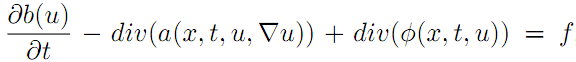,where the right side belongs to L1(Ω×(0,T)) and b(u) is unbounded function of u, the term div(a(x,t,u,u)) is a Leray--Lions operator and the function φ is a nonlinear lower order and satisfy only the growth condition.

1: Paper Source PDF document

Paper's Title:

A Dynamic Contact Problem for an Electro Viscoelastic Body

Author(s):

Denche M. and Ait Kaki L.

Laboratoire Equations Differentielles,
Departement de Mathematiques,
Universite Constantine 1,
Algeria.

Ecole Normale Superieure,
Departement des Sciences Exactes et Informatique,
Plateau Mansourah, Constantine.
Algeria.

Abstract:

We consider a dynamic problem which describes a contact between a piezoelectric body and a conductive foundation. The frictionless contact is modelled with the normal compliance, the electric conditions are supposed almost perfect. We prove the existence of a unique weak solution for almost perfect electric contact.

1: Paper Source PDF document

Paper's Title:

End-Point and Transversality Conditions in the Calculus of Variations: Derivations through Direct Reasoning

Author(s):

Olivier de La Grandville

Stanford University,
Department of Management Science and Engineering,
475 Via Ortega, Stanford, CA 94305,
U. S. A.

E-mail: ola@stanford.edu

Abstract:

We offer an intuitive explanation of the end-point and transversality conditions that complement the Euler equation in the calculus of variations. Our reasoning is based upon the fact that any variation given to an optimal function must entail a zero net gain to the functional, all consequences of implied changes in its derivative being fully taken into account.

1: Paper Source PDF document

Paper's Title:

Application of Equivalence Method to Classify Monge-Ampčre Equations of Elliptic Type

Author(s):

Moheddine Imsatfia

E-mail: imsatfia@math.jussieu.fr

Abstract:

In this paper, we apply Cartan's equivalence method to give a local classification of Monge-Ampčre equations of elliptic type. Then we find a necessary and sufficient conditions such that a Monge-Ampčre equation is either contactomorphic to the Laplace equation or to an Euler-Lagrange equation.

1: Paper Source PDF document

Paper's Title:

A Nonhomogeneous Subdiffusion Heat Equation

Author(s):

Joel-Arturo Rodriguez-Ceballos, Ana-Magnolia Marin, Ruben-Dario Ortiz

Instituto Tecnológico de Morelia,
Morelia, Michoacan,
Mexico

E-mail: joel@ifm.umich.mx

Campus San Pablo,
Cartagena de Indias, Bolivar,
Colombia

E-mail: amarinr@unicartagena.edu.co

E-mail:  joel@ifm.umich.mx

Abstract:

In this paper we consider a nonhomogeneous subdiffusion heat equation of fractional order with Dirichlet boundary conditions.

1: Paper Source PDF document

Paper's Title:

Asymptotic Analysis of Positive Decreasing Solutions of a Class of Systems of Second Order Nonlinear Differential Equations in the Framework of Regular Variation

Author(s):

Jaroslav Jaroš, Kusano Takaŝi, Tomoyuki Tanigawa

Department of Mathematical Analysis and Numerical Mathematics,
Faculty of Mathematics, Physics and Informatics,
Comenius Universiy, 842 48 Bratislava,
Slovakia.

E-mail: ksntksjm4@gmail.com

Professor Emeritus at: Hiroshima University,
Department of Mathematics, Faculty of Science,
Higashi-Hiroshima 739-8526,
Japan.

E-mail: jaros@fmph.uniba.sk

Department of Mathematics, Faculty of Education,
Kumamoto University, Kumamoto 860-8555,
Japan.

E-mail: tanigawa@educ.kumamoto-u.ac.jp

Abstract:

The system of nonlinear differential equations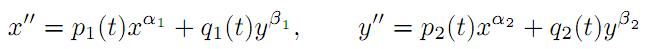is under consideration, where αi and βi are positive constants and pi(t) and qi(t) are continuous regularly varying functions on [a,). Two kinds of criteria are established for the existence of strongly decreasing regularly varying solutions with negative indices of (A) with precise asymptotic behavior at infinity. Fixed point techniques and basic theory of regular variation are utilized for this purpose.

1: Paper Source PDF document

Paper's Title:

Examples of Fractals Satisfying the Quasihyperbolic Boundary Condition

Author(s):

Petteri Harjulehto and Riku Klén

Department of Mathematics and Statistics,
FI-20014 University of Turku,
Finland

E-mail: petteri.harjulehto@utu.fi

E-mail: riku.klen@utu.fi

Abstract:

In this paper we give explicit examples of bounded domains that satisfy the quasihyperbolic boundary condition and calculate the values for the constants. These domains are also John domains and we calculate John constants as well. The authors do not know any other paper where exact values of parameters has been estimated.

1: Paper Source PDF document

Paper's Title:

Weak Type Inequalities for Some Operators on Generalized Morrey Spaces Over Metric Measure Spaces

Author(s):

Idha Sihwaningrum, Ari Wardayani, Hendra Gunawan

Faculty of Mathematics and Natural Sciences,
Jenderal Soedirman University, Purwokerto 53122,
Indonesia.
E-mail: idha.sihwaningrum@unsoed.ac.id ariwardayani@yahoo.co.id

Faculty of Mathematics and Natural Sciences,
Bandung Institute of Technology, Bandung 40132,
Indonesia.
E-mail: hgunawan@math.itb.ac.id
URL: http://personal.fmipa.itb.ac.id/hgunawan/

Abstract:

We discuss weak type inequalities for maximal and fractional integral operators on generalized Morrey spaces over metric measure spaces. Here the measure satisfies the so called growth condition. By taking into account the maximal operator, we obtain a Hedberg type inequality, which leads us to the weak type inequality for the fractional integral operator on the same spaces.

1: Paper Source PDF document

Paper's Title:

Some interesting properties of finite continuous Cesŕro operators

Author(s):

Abdelouahab Mansour and Abderrazak Hechifa

Operator theory laboratory (LABTHOP),
Eloued University,
Algeria.
E-mail: amansour@math.univ-lyon1.fr

Mathematics Department,
Faculty of Science,
Algeria.
E-mail: abderrazak02@gmail.com

Abstract:

A complex scalar λ is called an extended eigenvalue of a bounded linear operator T on a complex Banach space if there is a nonzero operator X such that TX = λ XT, the operator X is called extended eigenoperator of T corresponding to the extended eigenvalue λ.

In this paper we prove some properties of extended eigenvalue and extended eigenoperator for C1 on Lp([0,1]), where C1 is the Cesŕro operator defined on the complex Banach spaces Lp([0 , 1])  for 1<p<∞ by the expression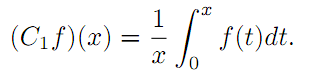1: Paper Source PDF document

Paper's Title:

Generalized Weighted Trapezoid and Grüss Type Inequalities on Time Scales

Author(s):

Eze Raymond Nwaeze

Department of Mathematics,
Tuskegee University,
Tuskegee, AL 36088,
USA.
E-mail: enwaeze@mytu.tuskegee.edu

Abstract:

In this work, we obtain some new generalized weighted trapezoid and Grüss type inequalities on time scales for parameter functions. Our results give a broader generalization of the results due to Pachpatte in . In addition, the continuous and discrete cases are also considered from which, other results are obtained.

1: Paper Source PDF document

Paper's Title:

On Interpolation of L2 functions

Author(s):

Anis Rezgui

Department of Mathematics,
Faculty of Sciences,
Taibah University, Al Madina Al Munawara,
KSA.

Mathematics Department,
INSAT,
University of Carthage, Tunis,
Tunisia
E-mail: anis.rezguii@gmail.com

Abstract:

In this paper we are interested in polynomial interpolation of irregular functions namely those elements of L2(R,μ) for μ a given probability measure. This is of course doesn't make any sense unless for L2 functions that, at least, admit a continuous version. To characterize those functions we have, first, constructed, in an abstract fashion, a chain of Sobolev like subspaces of a given Hilbert space H0. Then we have proved that the chain of Sobolev like subspaces controls the existence of a continuous version for L2 functions and gives a pointwise polynomial approximation with a quite accurate error estimation.

1: Paper Source PDF document

Paper's Title:

Wavelet Frames in Higher Dimensional Sobolev Spaces

Author(s):

Raj Kumar, Manish Chauhan, and Reena

Department of Mathematics,
Kirori Mal College, University of Delhi,
New Delhi-110007,
India.
E-mail: rajkmc@gmail.com

Department of Mathematics,
University of Delhi,
New Delhi-110007,
India
E-mail: manish17102021@gmail.com

Department of Mathematics,
Hans Raj College, University of Delhi,
New Delhi-110007,
India
E-mail: reena.bhagwat29@gmail.com

Abstract:

In this paper, we present sufficient condition for the sequence of vectors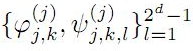to be a frame for Hs(Rd) are derived. Necessary and sufficient conditions for the sequence of vectorsto be tight wavelet frames in Hs(Rd) are obtained. Further, as an application an example of tight wavelet frames for Hs(R2) as bivariate box spline over 3-direction are given.

1: Paper Source PDF document

Paper's Title:

Mapped Chebyshev Spectral Methods for Solving Second Kind Integral Equations on the Real Line

Author(s):

Ahmed Guechi and Azedine Rahmoune

Department of Mathematics, University of Bordj Bou Arréridj,
El Anasser, 34030, BBA,
Algeria.
E-mail: a.guechi2017@gmail.com
E-mail: a.rahmoune@univ-bba.dz

Abstract:

In this paper we investigate the utility of mappings to solve numerically an important class of integral equations on the real line. The main idea is to map the infinite interval to a finite one and use Chebyshev spectral-collocation method to solve the mapped integral equation in the finite interval. Numerical examples are presented to illustrate the accuracy of the method.

1: Paper Source PDF document

Paper's Title:

Relation Between The Set Of Non-decreasing Functions And The Set Of Convex Functions

Author(s):

Qefsere Doko Gjonbalaj and Luigj Gjoka

Department of Mathematics, Faculty of Electrical and Computer Engineering,
University of Prishtina "Hasan Prishtina",
Prishtine 10000,
Kosova

E-mail: qefsere.gjonbalaj@uni-pr.edu

Department of Engineering Mathematics,
Polytechnic University of Tirana, Tirana,
Albania.

E-mail: luigjgjoka@ymail.com

Abstract:

In this article we address the problem of integral presentation of a convex function. Let I be an interval in R. Here, using the Riemann or Lebesgue’s integration theory, we find the necessary and sufficient condition for a function f: I R to be convex in I.

1: Paper Source PDF document

Paper's Title:

A Comparison Between Two Different Stochastic Epidemic Models with Respect to the Entropy

Author(s):

Department of Mathematics,
University of Sussex,
Brighton BN1 9QH,
UK.
E-mail: f.fatehi@sussex.ac.uk
URL: http://www.sussex.ac.uk/profiles/361251

Department of Pure Mathematics, Faculty of Mathematics and Computer,
Shahid Bahonar University of Kerman,
Kerman 76169-14111,
Iran.

Abstract:

In this paper at first a brief history of mathematical models is presented with the aim to clarify the reliability of stochastic models over deterministic models. Next, the necessary background about random variables and stochastic processes, especially Markov chains and the entropy are introduced. After that, entropy of SIR stochastic models is computed and it is proven that an epidemic will disappear after a long time. Entropy of a stochastic mathematical model determines the average uncertainty about the outcome of that random experiment. At the end, we introduce a chain binomial epidemic model and compute its entropy, which is then compared with the DTMC SIR epidemic model to show which one is nearer to reality.

1: Paper Source PDF document

Paper's Title:

Polynomial Dichotomy of C0-Quasi Semigroups in Banach Spaces

Author(s):

Sutrima1,2, Christiana Rini Indrati2, Lina Aryati2

1Department of Mathematics,
Universitas Sebelas Maret,
PO Box 57126, Surakarta,
Indonesia.
E-mail: sutrima@mipa.uns.ac.id

2Department of Mathematics,
PO Box 55281, Yogyakarta,
Indonesia.
E-mail: rinii@ugm.ac.id, lina@ugm.ac.id

Abstract:

Stability of solutions of the problems is an important aspect for application purposes. Since its introduction by Datko , the concept of exponential stability has been developed in various types of stability by various approaches. The existing conditions use evolution operator, evolution semigroup, and quasi semigroup approach for the non-autonomous problems and a semigroup approach for the autonomous cases. However, the polynomial stability based on C0-quasi semigroups has not been discussed in the references. In this paper we propose a new stability for C0-quasi semigroups on Banach spaces i.e the polynomial stability and polynomial dichotomy. As the results, the sufficient and necessary conditions for the polynomial and uniform polynomial stability are established as well as the sufficiency for the polynomial dichotomy. The results are also confirmed by the examples.

1: Paper Source PDF document

Paper's Title:

A Multivalued Version of the Radon-Nikodym Theorem, via the Single-valued Gould Integral

Author(s):

Domenico Candeloro1, Anca Croitoru2, Alina Gavriluţ2, Anna Rita Sambucini1

1Dept. of Mathematics and Computer Sciences,
University of Perugia,
1, Via Vanvitelli -- 06123, Perugia,
Italy.
E-mail:  domenico.candeloro@unipg.it, anna.sambucini@unipg.it

2Faculty of Mathematics,
Al. I. Cuza University,
700506 Iaşi,
Romania.
E-mail: croitoru@uaic.ro, gavrilut@uaic.ro

Abstract:

In this paper we consider a Gould type integral of real functions with respect to a compact and convex valued not necessarily additive measure. In particular we will introduce the concept of integrable multimeasure and, thanks to this notion, we will establish an exact Radon-Nikodym theorem relative to a fuzzy multisubmeasure which is new also in the finite dimensional case. Some results concerning the Gould integral are also obtained.

1: Paper Source PDF document

Paper's Title:

Convergence Speed of Some Random Implicit-Kirk-type Iterations for Contractive-type Random Operators

Author(s):

H. Akewe, K.S. Eke

Department of Mathematics,
Covenant University,
Canaanland, KM 10, Idiroko Road, P. M. B. 1023, Ota, Ogun State,
Nigeria.
E-mail: hudson.akewe@covenantuniversity.edu.ng, kanayo.eke@covenantuniversity.edu.ng

Abstract:

The main aim of this paper is to introduce a stochastic version of multistep type iterative scheme called a modified random implicit-Kirk multistep iterative scheme and prove strong convergence and stability results for a class of generalized contractive-type random operators. The rate of convergence of the random iterative schemes are also examined through an example. The results show that our new random implicit kirk multistep scheme perform better than other implicit iterative schemes in terms of convergence and thus have good potentials for further applications in equilibrium problems in computer science, physics and economics.

1: Paper Source PDF document

Paper's Title:

Characterization of Caristi Type Mapping Through its Absolute Derivative

Author(s):

M. Muslikh1, A. Kilicman2,3, S. H. Sapar4 and N. Bacho5

1Department of Mathematics,
University of Brawijaya,
Malang 65143, East Java,
Indonesia.
E-mail: mslk@ub.ac.id

2Department of Mathematics,
Universiti Putra Malaysia,
43400 UPM, Serdang, Selangor,
Malaysia
E-mail: akilic@upm.edu.my

3Department of Electrical and Electronic Engineering,
Istanbul Gelisim University,
Avcilar, Istanbul,
Turkey

4Department of Mathematics,
Universiti Putra Malaysia,
43400 UPM, Serdang, Selangor,
Malaysia
E-mail: sitihas@upm.edu.my

5Department of Mathematics,
Universiti Putra Malaysia,
43400 UPM, Serdang, Selangor,
Malaysia
E-mail: norfifah@upm.edu.my

Abstract:

The purpose of this article to characterize the Caristi type mapping by the absolute derivative. The equivalences of the Caristi mapping with contraction mapping is discussed too. In addition, it was shown that the contraction mapping can be tested through its absolute derivative.

1: Paper Source PDF document

Paper's Title:

Existence and Estimate of the Solution for the Approximate Stochastic Equation to the Viscous Barotropic Gas

Author(s):

R. Benseghir and A. Benchettah

LANOS Laboratory,
PO Box 12,
Annaba, Algeria.
E-mail: benseghirrym@ymail.com, abenchettah@hotmail.com

Abstract:

A stochastic equation of a viscous barotropic gas is considered. The application of Ito formula to a specific functional in an infinite dimensional space allows us to obtain an estimate which is useful to analyse the behavior of the solution. As it is difficult to exploit this estimate, we study an approximate problem. More precisely, we consider the equation of a barotropic viscous gas in Lagrangian coordinates and we add a diffusion of the density. An estimate of energy is obtained to analyse the behavior of the solution for this approximate problem and Galerkin method is used to prove the existence and uniqueness of the solution.

1: Paper Source PDF document

Paper's Title:

An Existence of the Solution to Neutral Stochastic Functional Differential Equations Under the Holder Condition

Author(s):

Young-Ho Kim

Department of Mathematics,
Changwon National University,
Changwon, Gyeongsangnam-do 51140,
Korea.

E-mail: iyhkim@changwon.ac.kr

Abstract:

In this paper, we show the existence and uniqueness of solution of the neutral stochastic functional differential equations under weakened H\"{o}lder condition, a weakened linear growth condition, and a contractive condition. Furthermore, in order to obtain the existence of a solution to the equation we used the Picard sequence.

1: Paper Source PDF document

Paper's Title:

Kinematic Model for Magnetic Null-points in 2 Dimensions

Author(s):

Ali Khalaf Hussain Al-Hachami

Department of Mathematics,
College of Education For Pure Sciences,
Wasit University,
Iraq.
E-mail: alhachamia@uowasit.edu.iq

Abstract:

The adjacent configurations of two-dimensional magnetic null point centers are analyzed by an immediate examination about the null. The configurations are classified as either potential or non-potential. By then the non-potential cases are subdivided into three cases depending upon whether the component of current is less than, equal to or greater than a threshold current. In addition the essential structure of reconnection in 2D is examined. It unfolds that the manner by which the magnetic flux is rebuilt. In this paper, we center on the ramifications of kinematic arrangements; that is, we fathom just Maxwell's conditions and a resistive Ohm's law.

1: Paper Source PDF document

Paper's Title:

Global Analysis on Riemannian Manifolds

Author(s):

Louis Omenyi and Michael Uchenna

Department of Mathematics, Computer Science, Statistics and Informatics,
Alex Ekwueme Federal University, Ndufu-Alike,
Nigeria.
E-mail: omenyi.louis@funai.edu.ng, michael.uchenna@funai.edu.ng
URL: http://www.funai.edu.ng

Abstract:

In this paper, an exposition of the central concept of global analysis on a Riemannan manifold is given. We extend the theory of smooth vector fields from open subsets of Euclidean space to Riemannan manifolds. Specifically, we prove that a Riemannian manifold admits a unique solution for a system of ordinary differential equations generated by the flow of smooth tangent vectors. The idea of partial differential equations on Riemannian manifold is highlighted on the unit sphere.

1: Paper Source PDF document

Paper's Title:

Generalised Models for Torsional Spine Reconnection

Author(s):

Ali Khalaf Hussain Al-Hachami

Department of Mathematics,
College of Education For Pure Sciences,
Wasit University,
Iraq.
E-mail: alhachamia@uowasit.edu.iq

Abstract:

Three-dimensional (3D) null points are available in wealth in the solar corona, and the equivalent is probably going to be valid in other astrophysical situations. On-going outcomes from sun oriented perceptions and from reproductions propose that reconnection at such 3D nulls may assume a significant job in the coronal dynamics. The properties of the torsional spine method of magnetic reconnection at 3D nulls are researched. Kinematic model are created, which incorporate the term ηJ that is spatially localised around the null, stretching out along the spine of the null. The null point is to research the impact of shifting the level of asymmetry of the null point magnetic field on the subsequent reconnection process where past examinations constantly considered a non-nonexclusive radially symmetric null. Specifically we analyse the rate of reconnection of magnetic flux at the spine of null point. Logical arrangements are determined for the enduring kinematic equation, and contrasted and the after effects of torsional spine reconnection models when the current is restricted in which the Maxwell conditions are illuminated. The geometry of the current layers inside which torsional spine reconnection happen is autonomous on the symmetry of the magnetic field. Torsional spine reconnection happens in a thin cylinder around the spine, with circular cross-segment when the fan eigenvalues are extraordinary. The short axis of the circle being along the solid field bearing. Just as it was discovered that the fundamental structure of the method of attractive reconnection considered is unaffected by changing the magnetic field symmetry, that is, the plasma flow is discovered rotational around the spine of null point. The spatiotemporal pinnacle current, and the pinnacle reconnection rate achieved, are found not to rely upon the level of asymmetry.

1: Paper Source PDF document

Paper's Title:

Applications of Von Neumann Algebras to Rigidity Problems of (2-Step) Riemannian (Nil-)Manifolds

Author(s):

DFouman Faculty of Engineering,
College of Engineering, University of Tehran,
Iran.

Department of Mathematical Sciences,
Sharif University of Technology,
Iran
E-mail: fanai@sharif.edu

Abstract:

In this paper, basic notions of von Neumann algebra and its direct analogues in the realm of groupoids and measure spaces have been considered. By recovering the action of a locally compact Lie group from a crossed product of a von Neumann algebra, other proof of one of a geometric propositions of O'Neil and an extension of it has been proposed. Also, using the advanced exploration of nilmanifolds in measure spaces and their corresponding automorphisms (Lie algebraic derivations) a different proof of an analytic theorem of Gordon and Mao has been attained. These two propositions are of the most important ones for rigidity problems of Riemannian manifolds especially 2-step nilmanifolds.

1: Paper Source PDF document

Paper's Title:

Double Difference of Composition Operator on Bloch Spaces

Author(s):

Rinchen Tundup

Department of Mathematics
University of Jammu
Jammu and Kashmir
India.

E-mail: joneytun123@gmail.com

Abstract:

In this paper we characterize the compactness of double difference of three non-compact composition operators on Bloch space induced by three holomorphic self maps on the unit disc.

1: Paper Source PDF document

Paper's Title:

Accuracy of Implicit DIMSIMs with Extrapolation

Author(s):

A. J. Kadhim, A. Gorgey, N. Arbin and N. Razali

Mathematics Department, Faculty of Science and Mathematics,
Sultan Idris Education University,
35900 Tanjong Malim, Perak,
Malaysia
E-mail: annie_gorgey@fsmt.upsi.edu.my

Unit of Fundamental Engineering Studies,
Faculty of Engineering, Built Environment,
The National University of Malaysia,
43600 Bangi,
Malaysia.

Abstract:

The main aim of this article is to present recent results concerning diagonal implicit multistage integration methods (DIMSIMs) with extrapolation in solving stiff problems. Implicit methods with extrapolation have been proven to be very useful in solving problems with stiff components. There are many articles written on extrapolation of Runge-Kutta methods however fewer articles on extrapolation were written for general linear methods. Passive extrapolation is more stable than active extrapolation as proven in many literature when solving stiff problems by the Runge-Kutta methods. This article takes the first step by investigating the performance of passive extrapolation for DIMSIMs type-2 methods. In the variable stepsize and order codes, order-2 and order-3 DIMSIMs with extrapolation are investigated for Van der Pol and HIRES problems. Comparisons are made with ode23 solver and the numerical experiments showed that implicit DIMSIMs with extrapolation has greater accuracy than the method itself without extrapolation and ode23.

1: Paper Source PDF document

Paper's Title:

Attempts to Define a Baum--Connes Map Via Localization of Categories for Inverse Semigroups

Author(s):

Bernhard Burgstaller

Departamento de Matematica,
CEP 88.040-900 Florianopolis-SC,
Brasil.
E-mail: bernhardburgstaller@yahoo.de
URL: http://mathematik.work/bernhardburgstaller/index.html

Abstract:

An induction functor in inverse semigroup equivariant KK-theory is considered, and together with %a restriction functors certain results similar to those known from the Mackey machinery are shown. It is also verified that for any so-called E-continuous inverse semigroup its equivariant KK-theory satisfies the universal property and is a triangulated category.

1: Paper Source PDF document

Paper's Title:

On The Degree of Approximation of Periodic Functions from Lipschitz and Those from Generalized Lipschitz Classes

Author(s):

Xhevat Z. Krasniqi

Faculty of Education,
University of Prishtina "Hasan Prishtina",
Avenue "Mother Theresa " no. 5, Prishtin
ë 10000,
Republic of Kosovo.
E-mail: xhevat.krasniqi@uni-pr.edu

Abstract:

In this paper we have introduced some new trigonometric polynomials. Using these polynomials, we have proved some theorems which determine the degree of approximation of periodic functions by a product of two special means of their Fourier series and the conjugate Fourier series. Many results proved previously by others are special case of ours.

1: Paper Source PDF document

Paper's Title:

Numerical Approximation by the Method of Lines with Finite-volume Approach of a Solute Transport Equation in Periodic Heterogeneous Porous Medium

Author(s):

D. J. Bambi Pemba and B. Ondami

Université Marien Ngouabi,
Factuté des Sciences et Techniques,
BP 69, Brazzaville,
Congo.
E-mail: bondami@gmail.com

Abstract:

In this paper we are interested in the numerical approximation of a two-dimensional solute transport equation in heterogeneous porous media having periodic structures. It is a class of problems which has been the subject of various works in the literature, where different methods are proposed for the determination of the so-called homogenized problem. We are interested in this paper, in the direct resolution of the problem, and we use the method of lines with a finite volume approach to discretize this equation. This discretization leads to an ordinary differential equation (ODE) that we discretize by the Euler implicit scheme. Numerical experiments comparing the obtained solution and the homogenized problem solution are presented. They show that the precision and robustness of this method depend on the ratio between, the mesh size and the parameter involved in the periodic homogenization.

1: Paper Source PDF document

Paper's Title:

Residual-Based A Posteriori Error Estimates For A Conforming Mixed Finite Element Discretization of the Monge-Ampere Equation

Author(s):

J. Adetola, K. W. Houedanou and B. Ahounou

Institut de Mathematiques et de Sciences Physiques (IMSP),
Universite d'Abomey-Calavi

Departement de Mathematiques,
Faculte des Sciences et Techniques (FAST),
Universite d'Abomey-Calavi
E-mail: khouedanou@yahoo.fr

Departement de Mathematiques,
Faculte des Sciences et Techniques (FAST),
Universite d'Abomey-Calavi
E-mail: bahounou@yahoo.fr

Abstract:

In this paper we develop a new a posteriori error analysis for the Monge-Ampere equation approximated by conforming finite element method on isotropic meshes in R2. The approach utilizes a slight variant of the mixed discretization proposed by Gerard Awanou and Hengguang Li in . The a posteriori error estimate is based on a suitable evaluation on the residual of the finite element solution. It is proven that the a posteriori error estimate provided in this paper is both reliable and efficient.

1: Paper Source PDF document

Paper's Title:

Reduced Generalized Combination Synchronization Between Two n-Dimensional Integer-Order Hyperchaotic Systems and One m-Dimensional Fractional-Order Chaotic System

Author(s):

Smail Kaouache, Mohammed Salah Abdelouahab and Rabah Bououden

Laboratory of Mathematics and their interactions,
Abdelhafid Boussouf University Center, Mila.
Algeria
E-mail: smailkaouache@gmail.com, medsalah3@yahoo.fr, rabouden@yahoo.fr

Abstract:

This paper is devoted to investigate the problem of reduced generalized combination synchronization (RGCS) between two n-dimensional integer-order hyperchaotic drive systems and one m-dimensional fractional-order chaotic response system. According to the stability theorem of fractional-order linear system, an active mode controller is proposed to accomplish this end. Moreover, the proposed synchronization scheme is applied to synchronize three different chaotic systems, which are the Danca hyperchaotic system, the modified hyperchaotic Rossler system, and the fractional-order Rabinovich-Fabrikant chaotic system. Finally, numerical results are presented to fit our theoretical analysis.

1: Paper Source PDF document

Paper's Title:

Sharp Inequalities Between Hölder and Stolarsky Means of Two Positive Numbers

Author(s):

M. Bustos Gonzalez and A. I. Stan

The University of Iowa,
Department of Mathematics,
14 MacLean Hall,
Iowa City, Iowa,
USA.
E-mail: margarita-bustosgonzalez@uiowa.edu

The Ohio State University at Marion,
Department of Mathematics,
1465 Mount Vernon Avenue,
Marion, Ohio,
USA.
E-mail: stan.7@osu.edu

Abstract:

Given any index of the Stolarsky means, we find the greatest and least indexes of the H\"older means, such that for any two positive numbers, the Stolarsky mean with the given index is bounded from below and above by the Hölder means with those indexes, of the two positive numbers. Finally, we present a geometric application of this inequality involving the Fermat-Torricelli point of a triangle.

1: Paper Source PDF document

Paper's Title:

Pointwise Convergence of Fourier-type Series with Exponential Weights

Author(s):

Hee Sun Jung and Ryozi Sakai

Department of Mathematics Education, Sungkyunkwan University,
Seoul 110-745,
Republic of Korea.
E-mail: hsun90@skku.edu

Department of Mathematics,
Meijo University, Nagoya 468-8502,
Japan.
E-mail: ryozi@hm.aitai.ne.jp

Abstract:

Let R = ( - ∞,∞), and let Q∈C1(R):R→[0,∞) be an even function. We consider the exponential weights w(x)=e-Q(x), xR. In this paper we obtain a pointwise convergence theorem for the Fourier-type series with respect to the orthonormal polynomials {pn(w2;x)}.

1: Paper Source PDF document

Paper's Title:

Semivectorial Bilevel Optimization on Affine-Finsler-Metric Manifolds

Author(s):

Faik Mayah1, Ali S Rasheed2 and Naseif J. Al- Jawari3

1Department of Physics,
College of Sciences,
University of Wasit,
Iraq.
E-mail: faik.mayah@gmail.com

2Ministry of Higher Education and Scientific Research,
Iraq.
E-mail: ali.math2018@yahoo.com ahmedhashem@gmail.com

3Dept. of Mathematics,
College of Science,